# RD Sharma Solutions for Class 8 Maths Chapter 14 Compound Interest

We have learnt about simple interest and the formula for calculating simple interest and amount in Class 7. Now in Chapter 14 of Class 8, we shall discuss concepts based on compound interest and the methods to calculate the compound interest. We shall also discuss problems on population growth and depreciation of the value of different assets. RD Sharma Class 8 is developed by our expert tutors aiming to help students learn the correct methods of solving problems. In order to strengthen the weaker areas in solving problems, make use of RD Sharma solutions which are provided here, to achieve your goals. Students can download the PDFs for free from the links provided below.

Chapter 14- Compound Interest contains five exercises and the RD Sharma Solutions present in this page provide solutions to the questions present in each exercise. Now, let us have a look at the concepts discussed in this chapter.

• Computation of compound interest – compounded annually, half-yearly and quarterly.
• Computation of compound interest by using Formulae.
• Inverse problems on compound interest.
• Population growth problems.
• Depreciation related problems.

## Download the Pdf of RD Sharma Solutions for Class 8 Maths Chapter 14 Compound Interest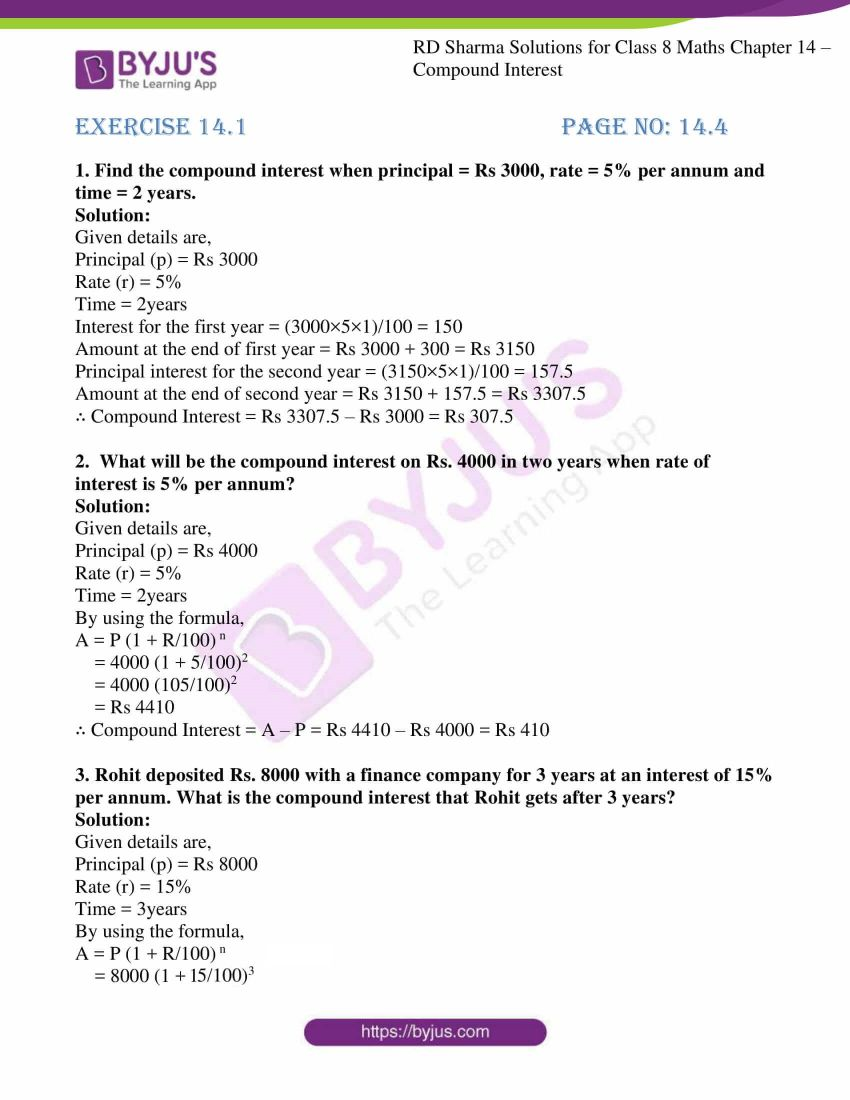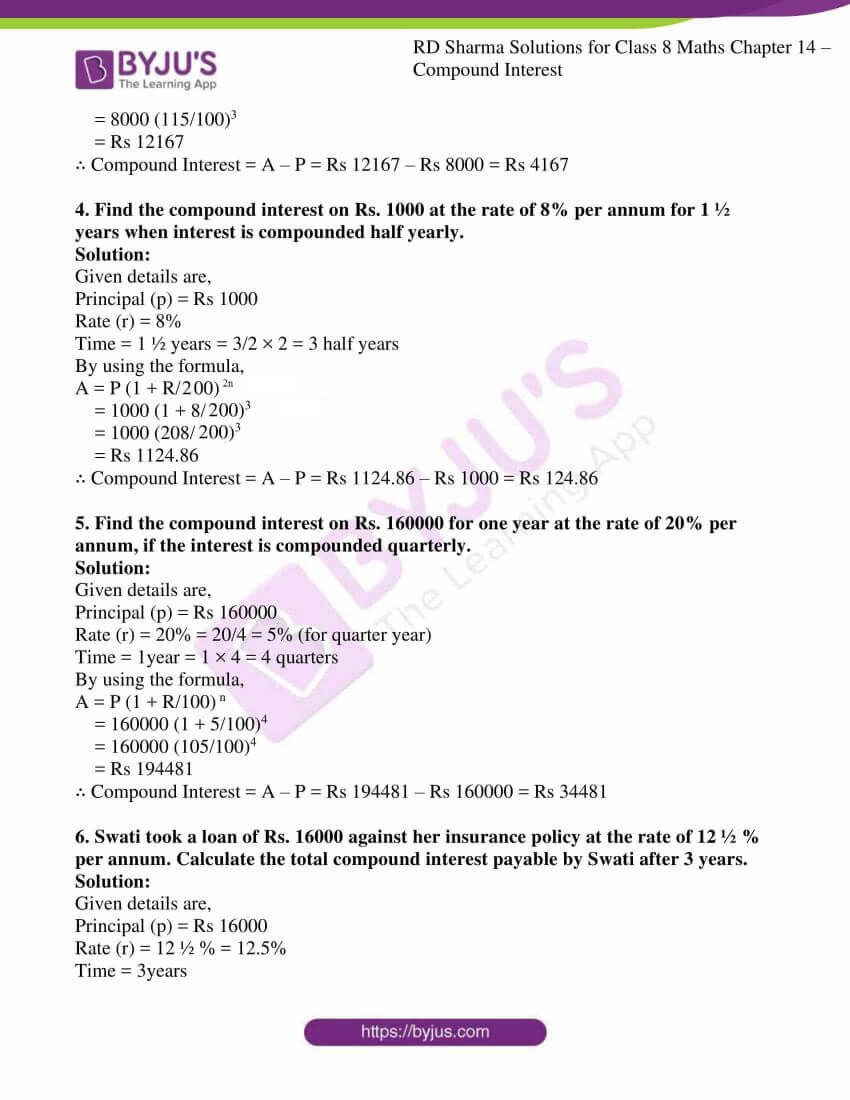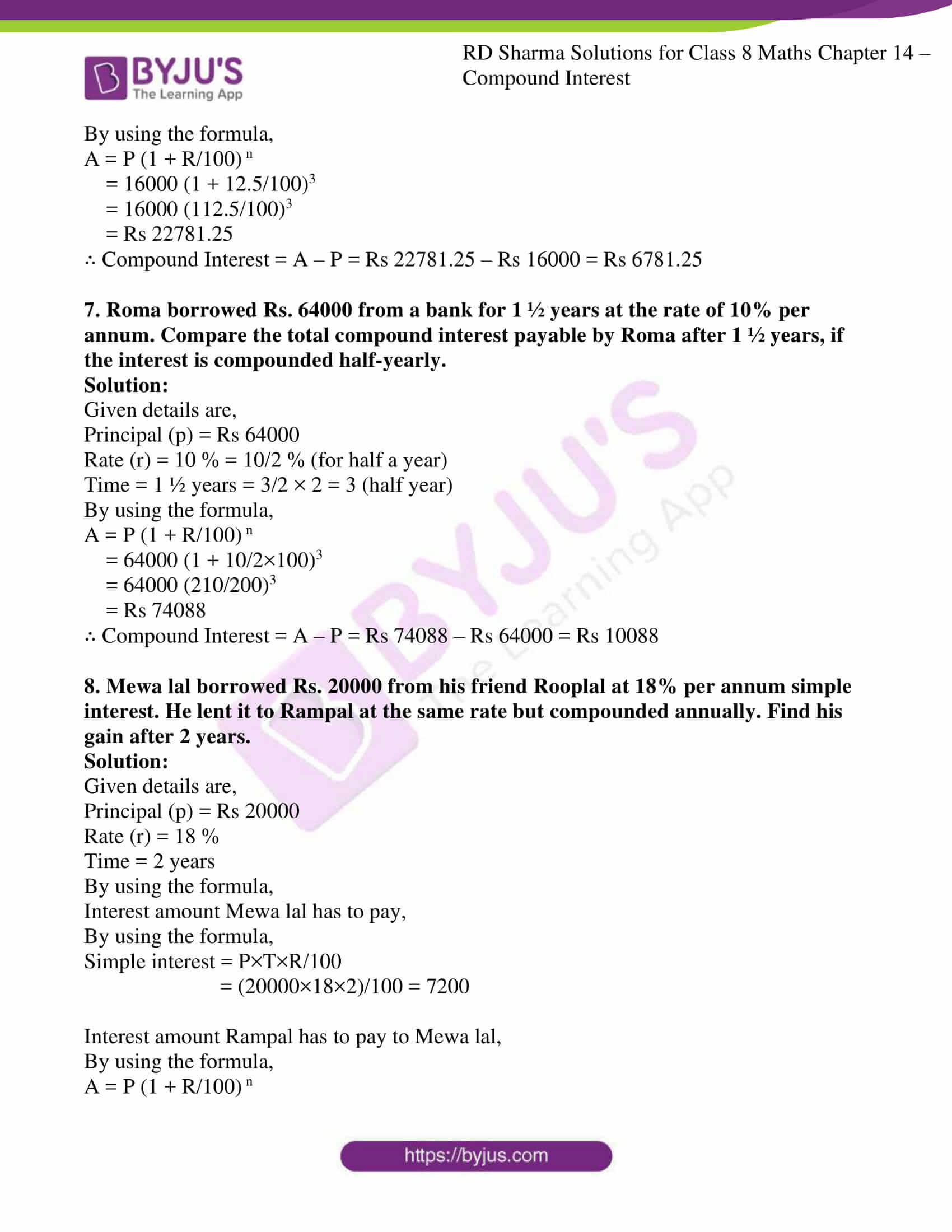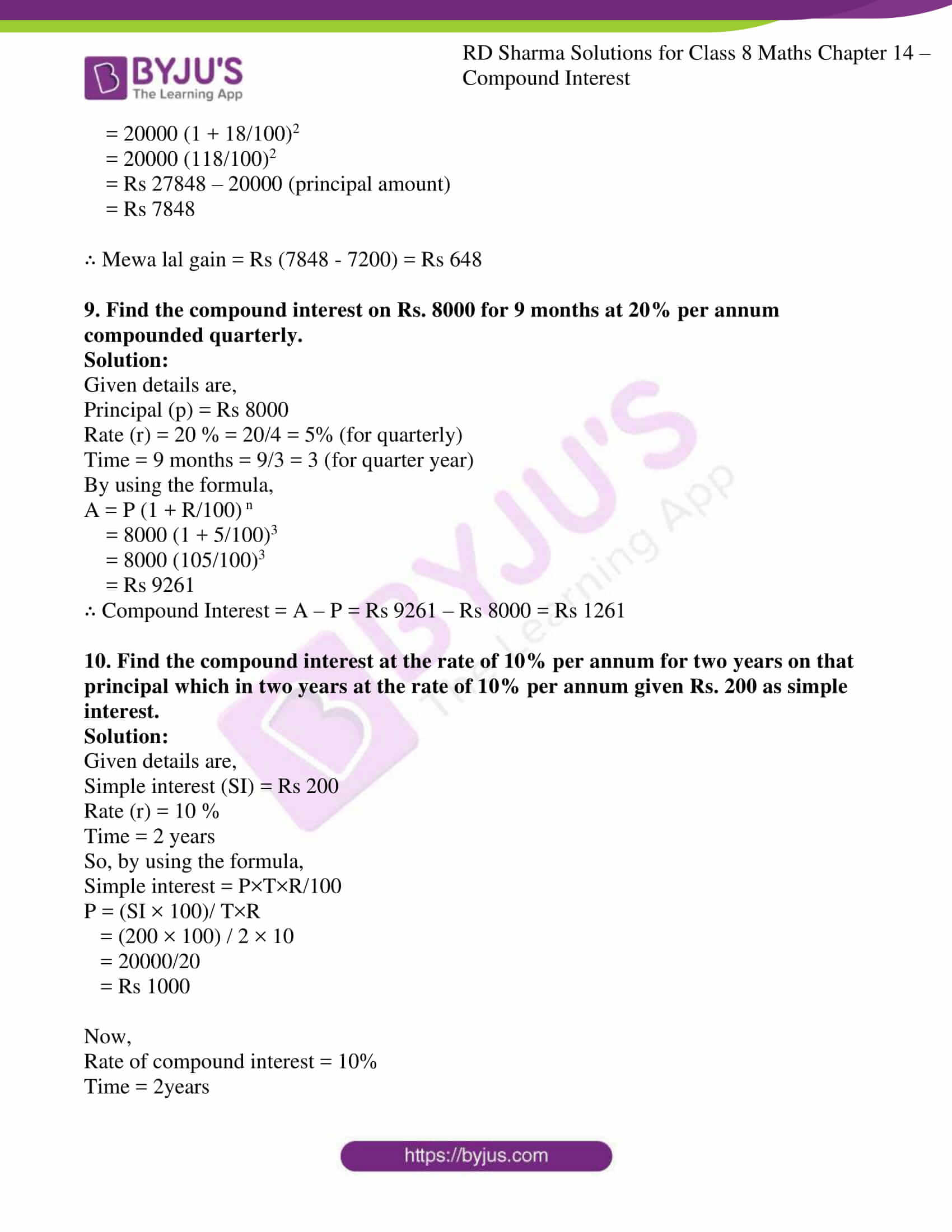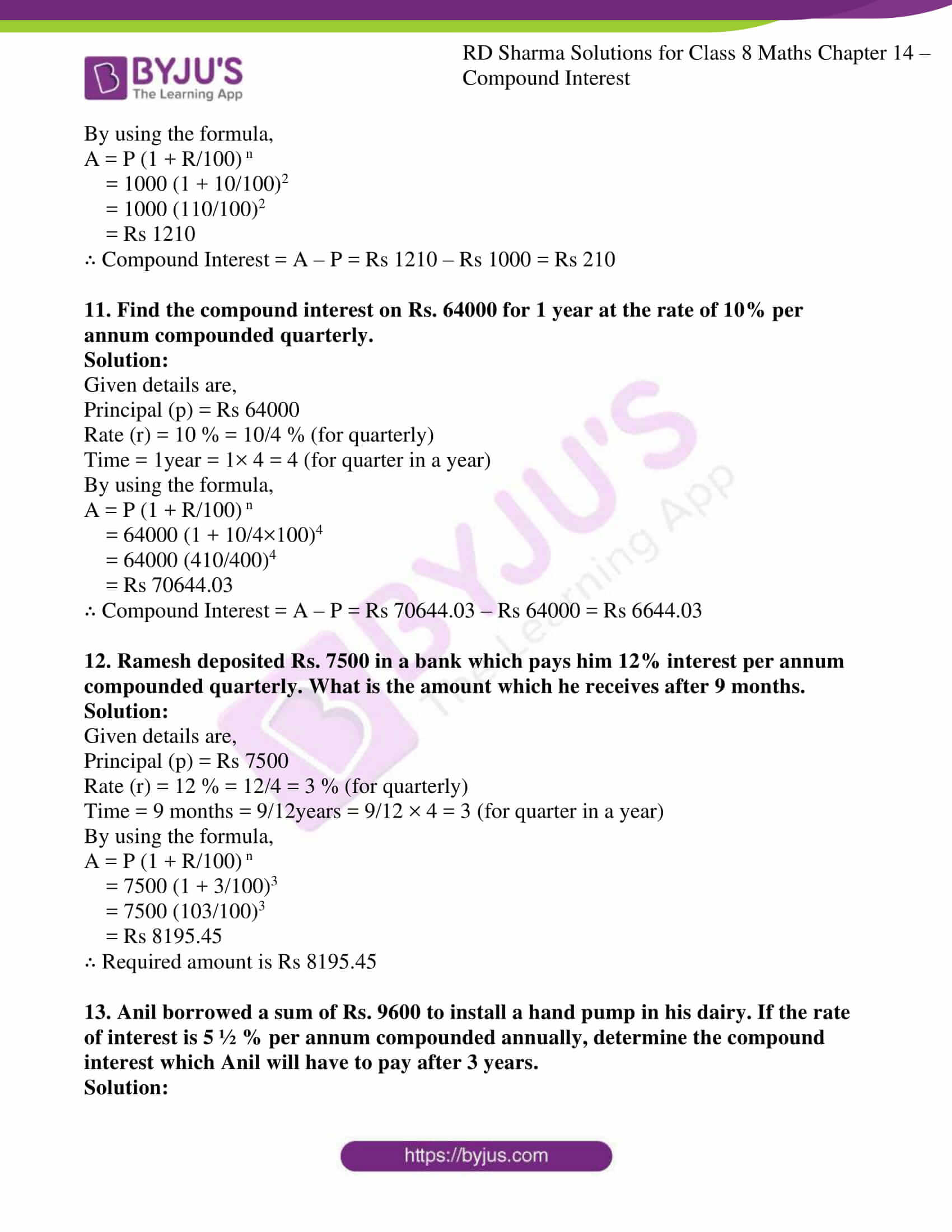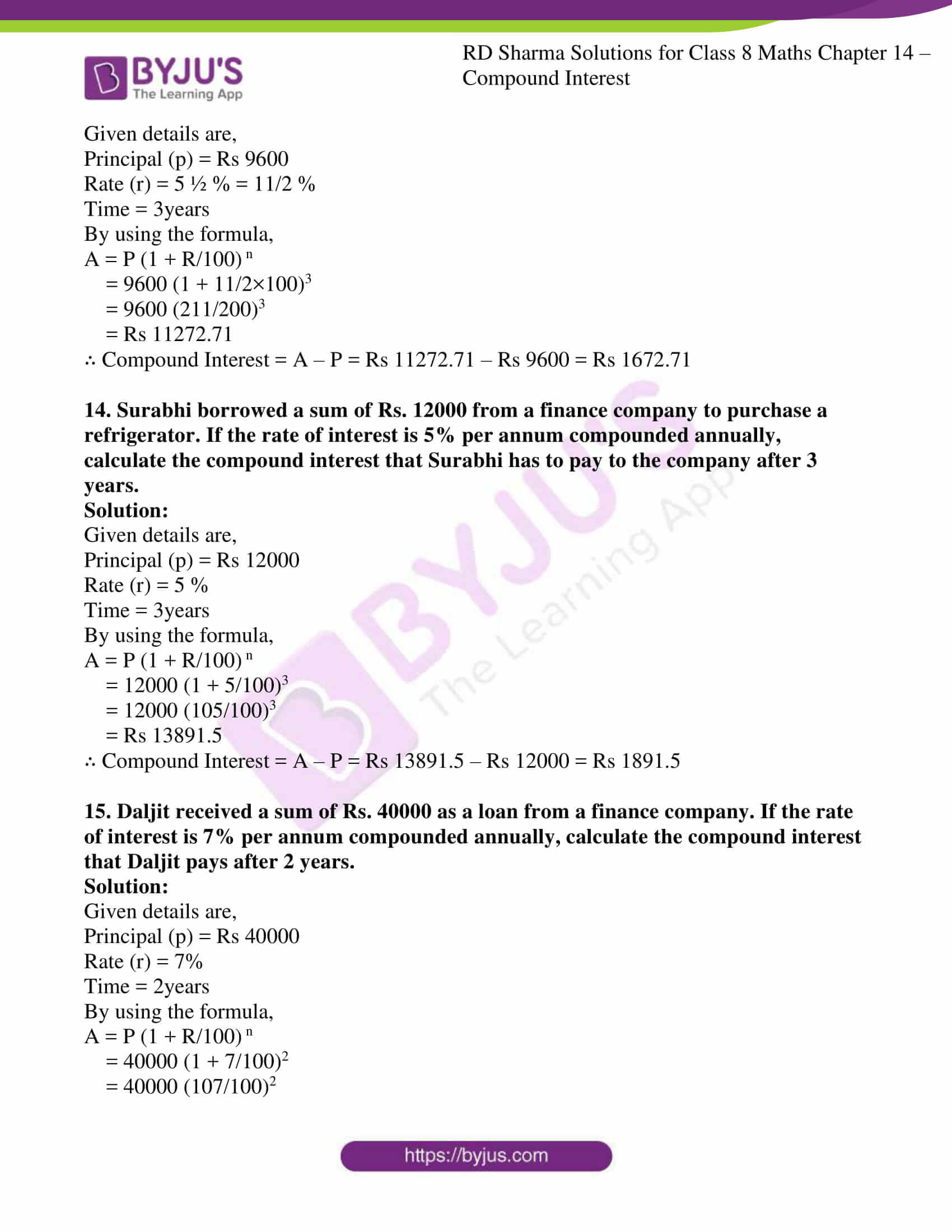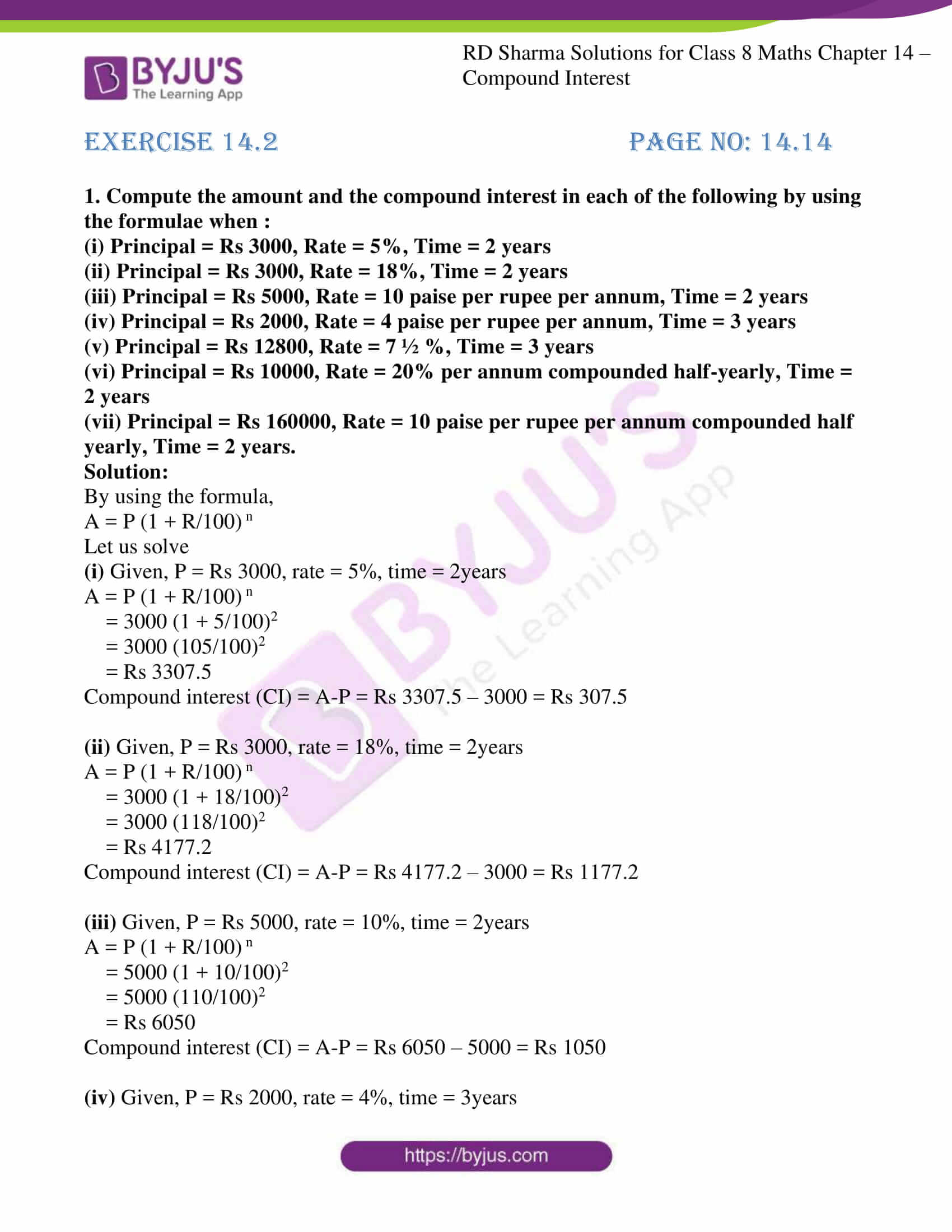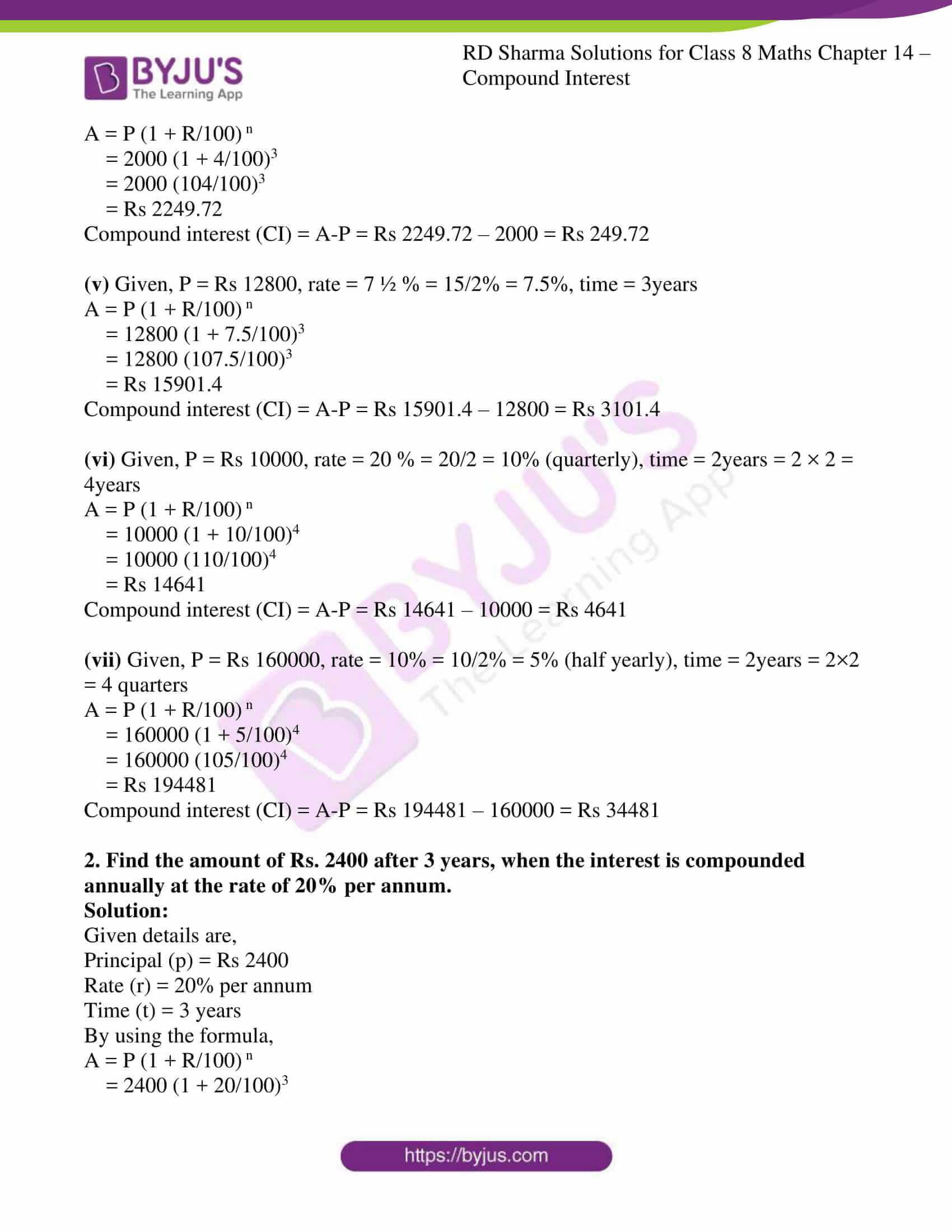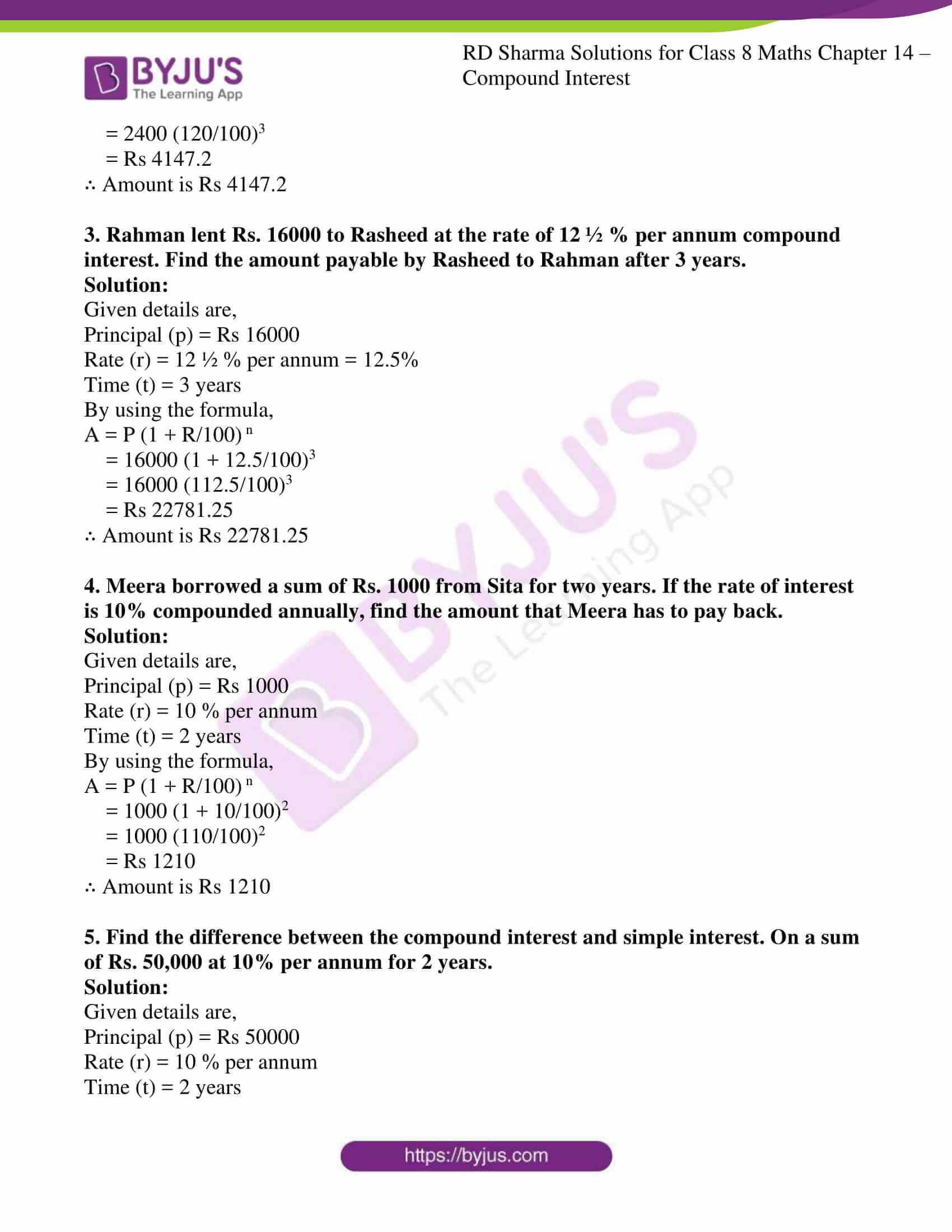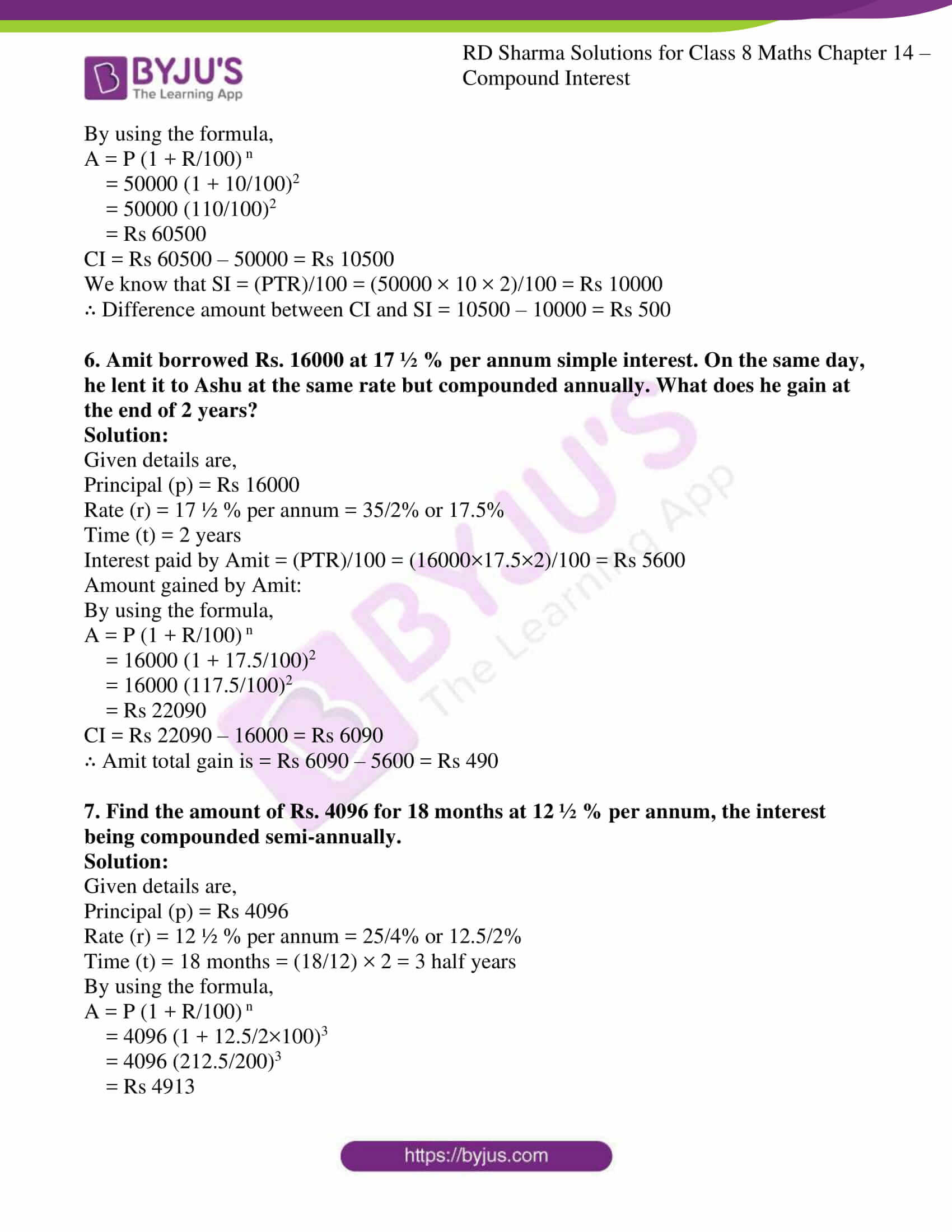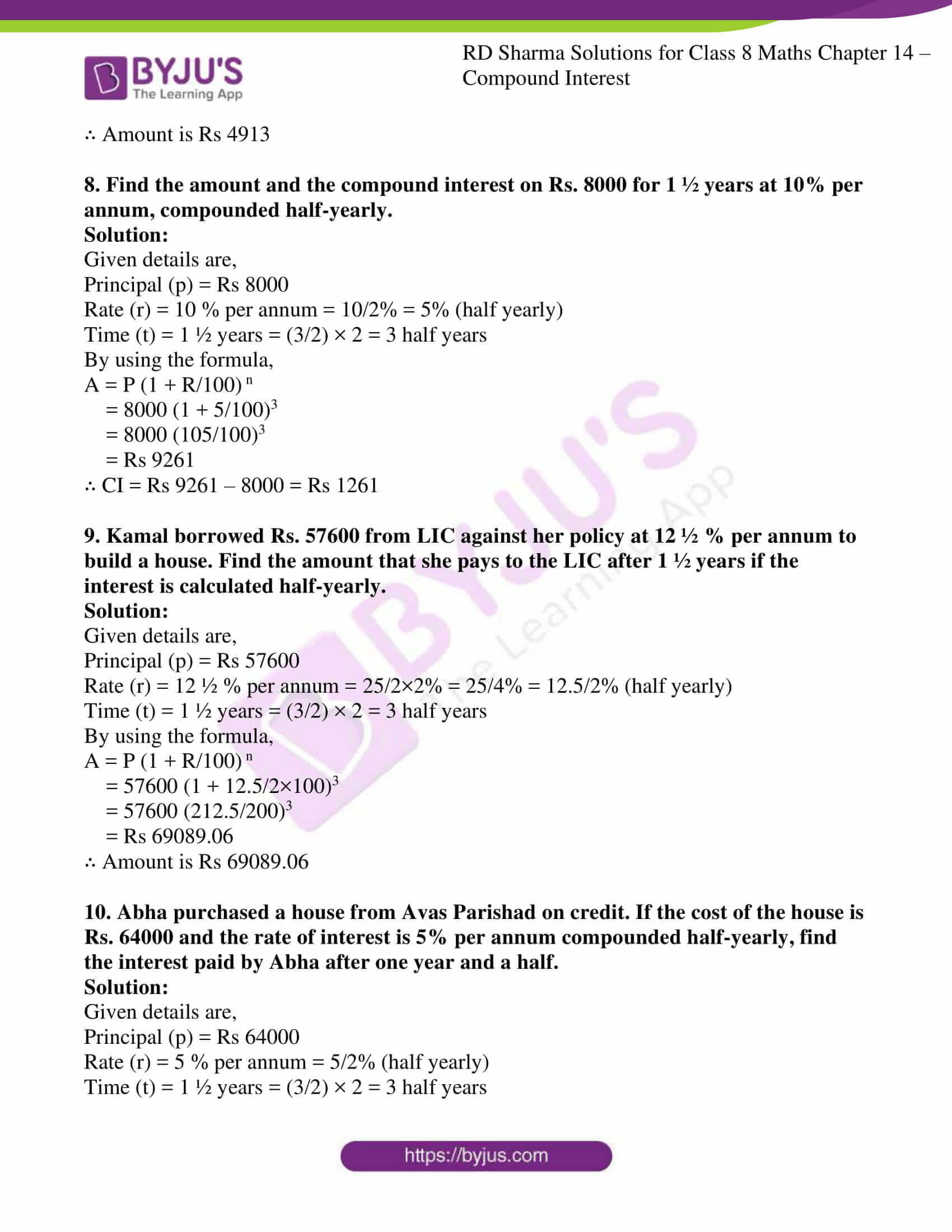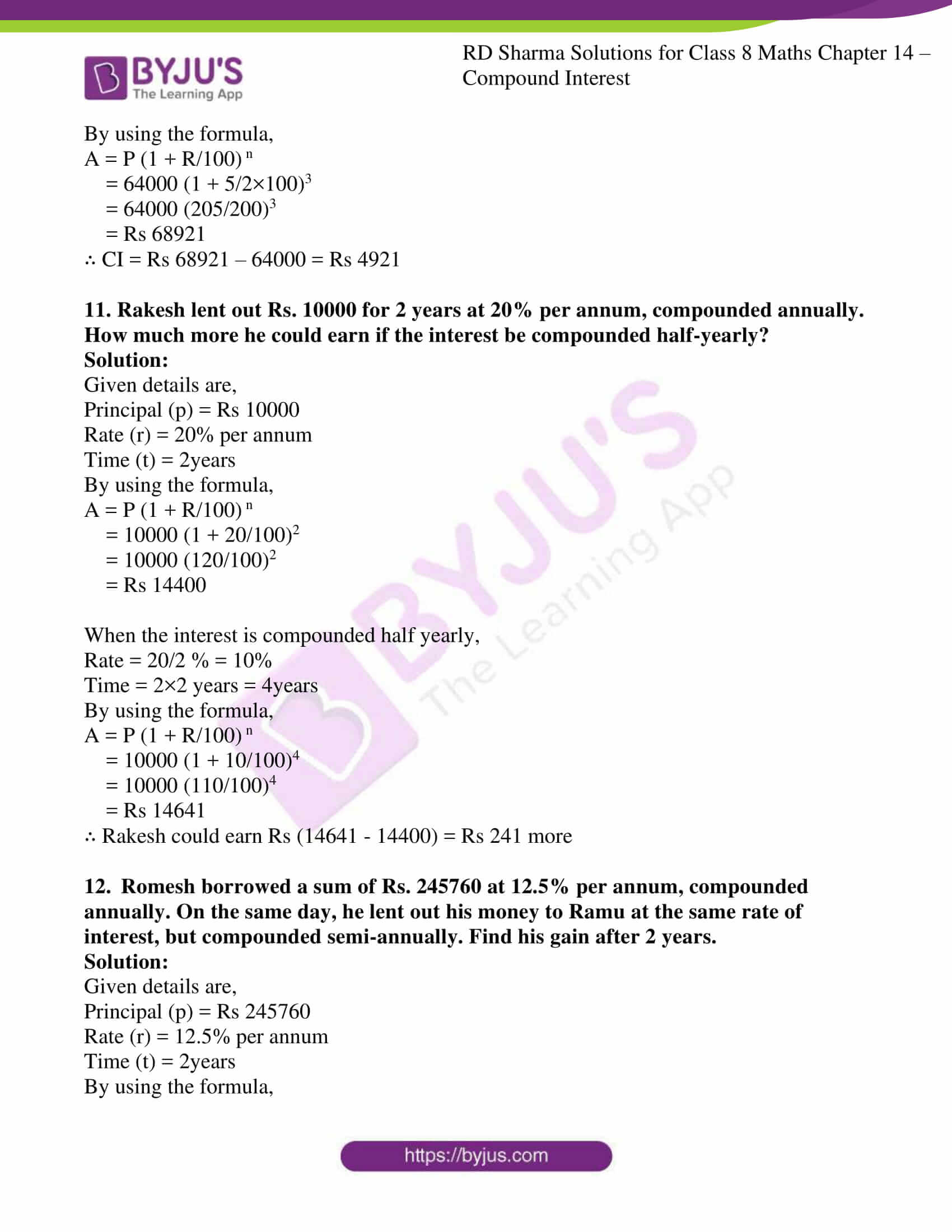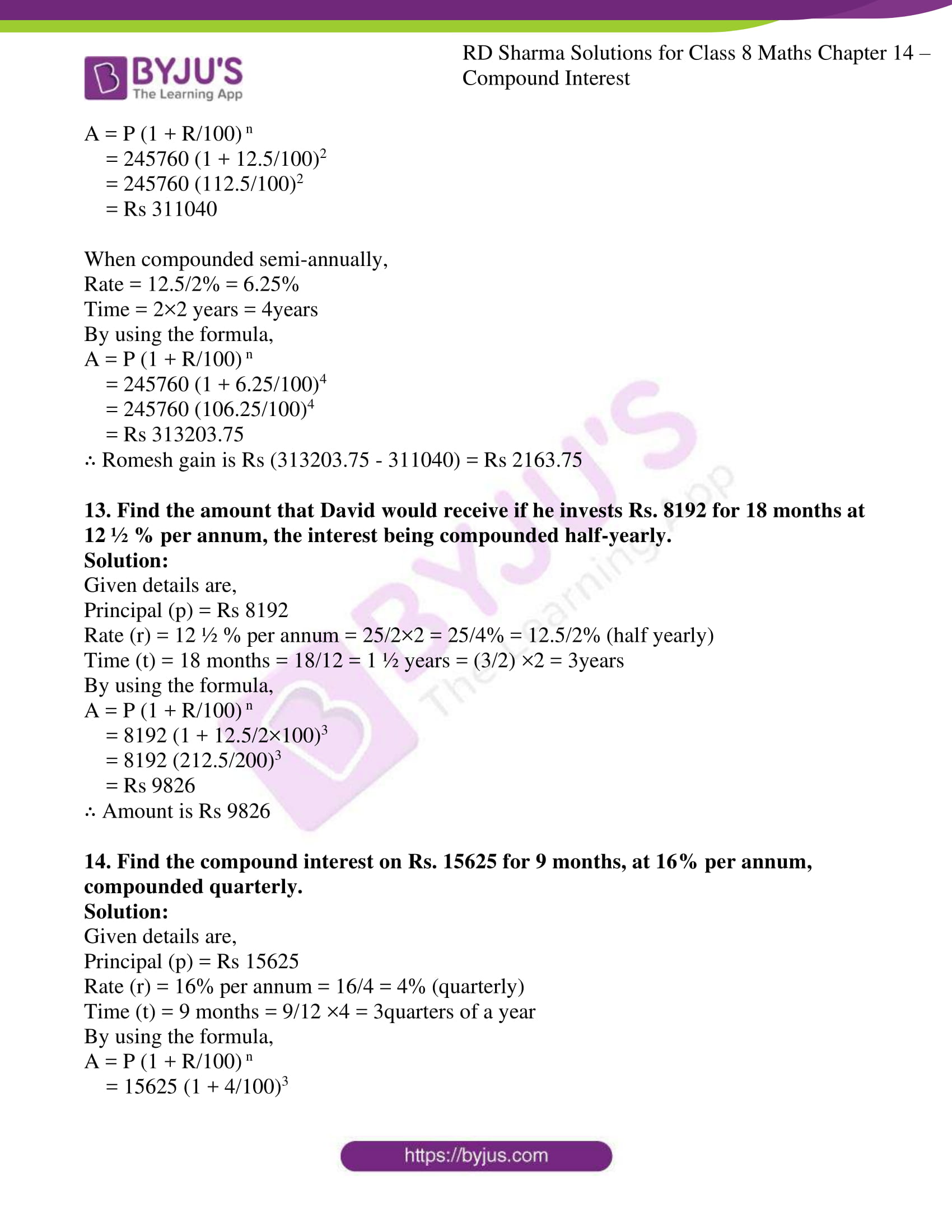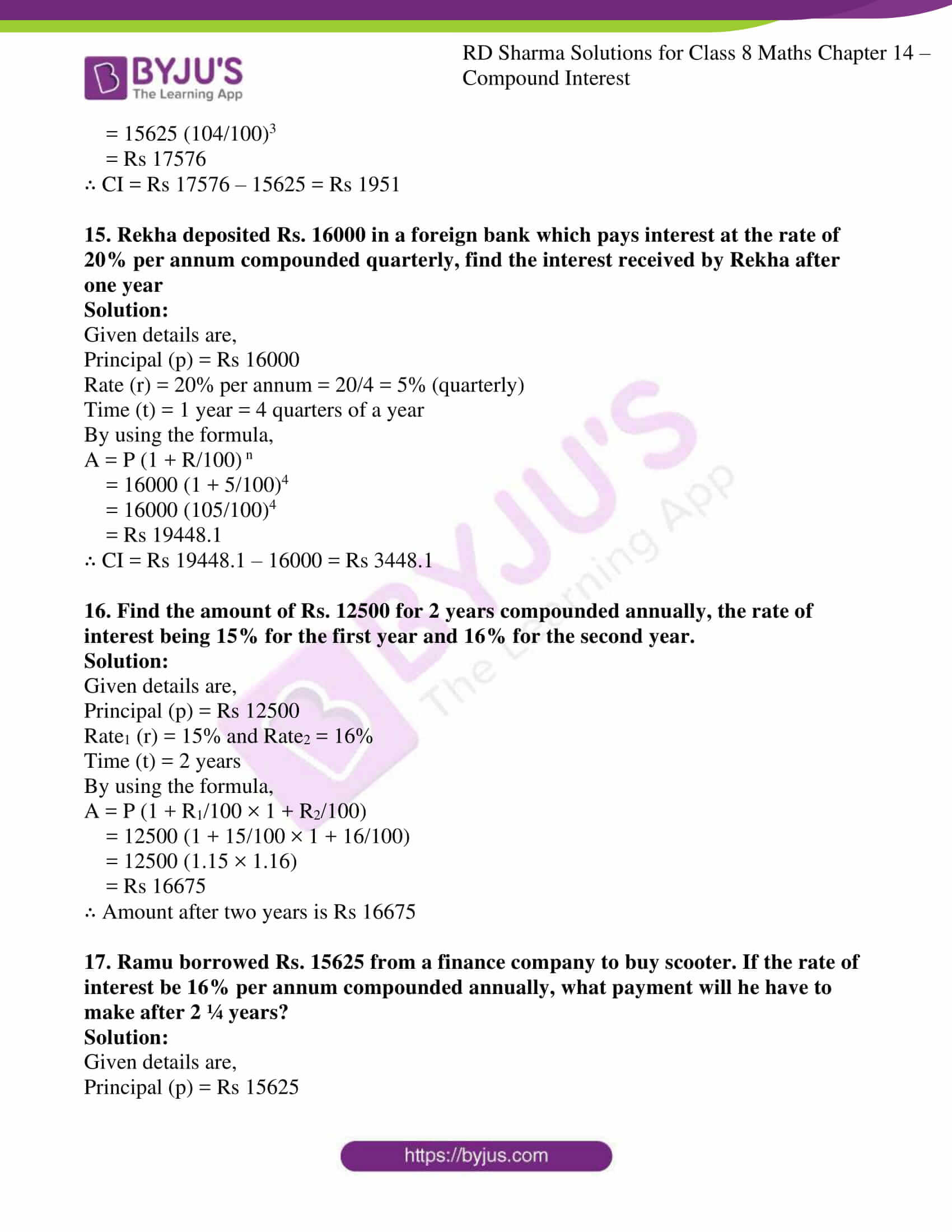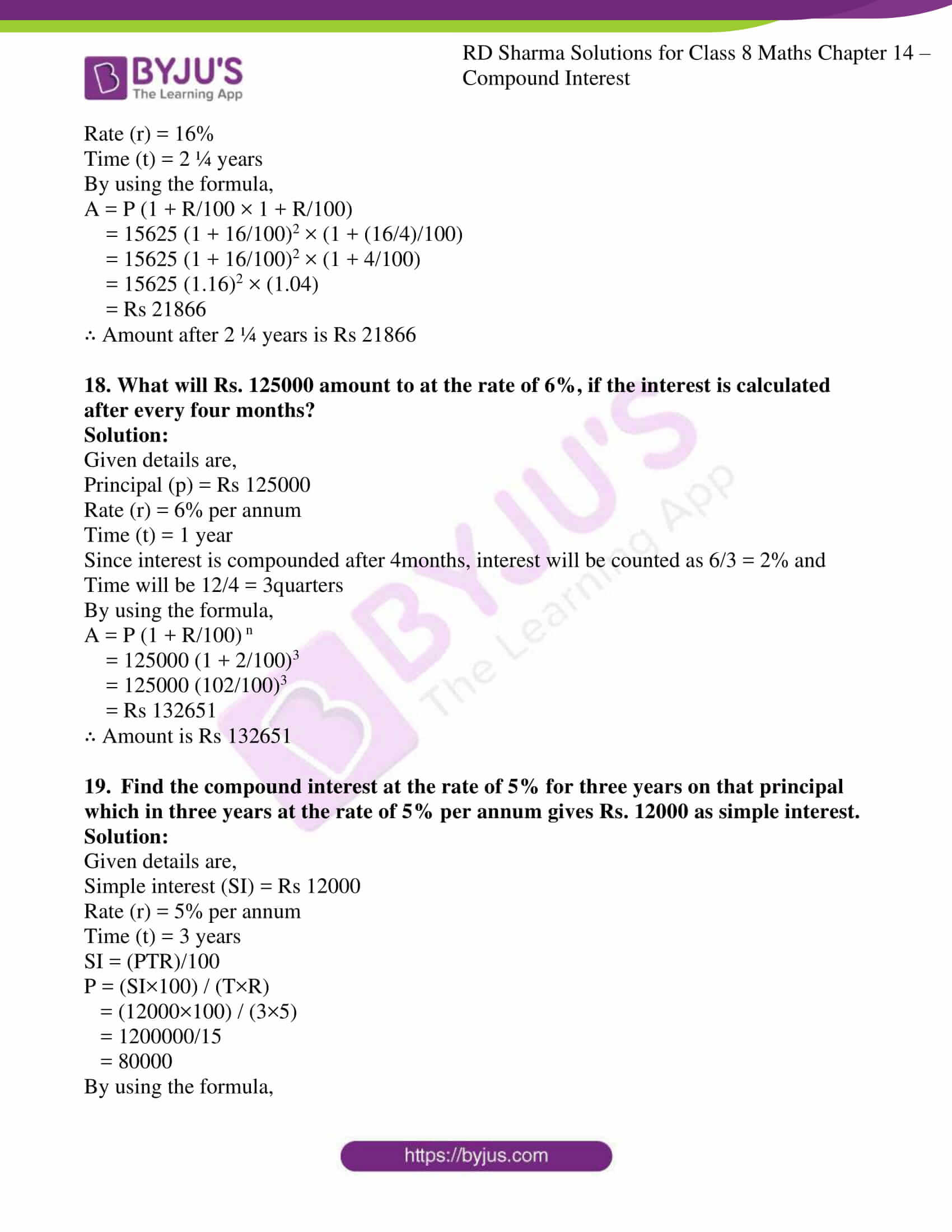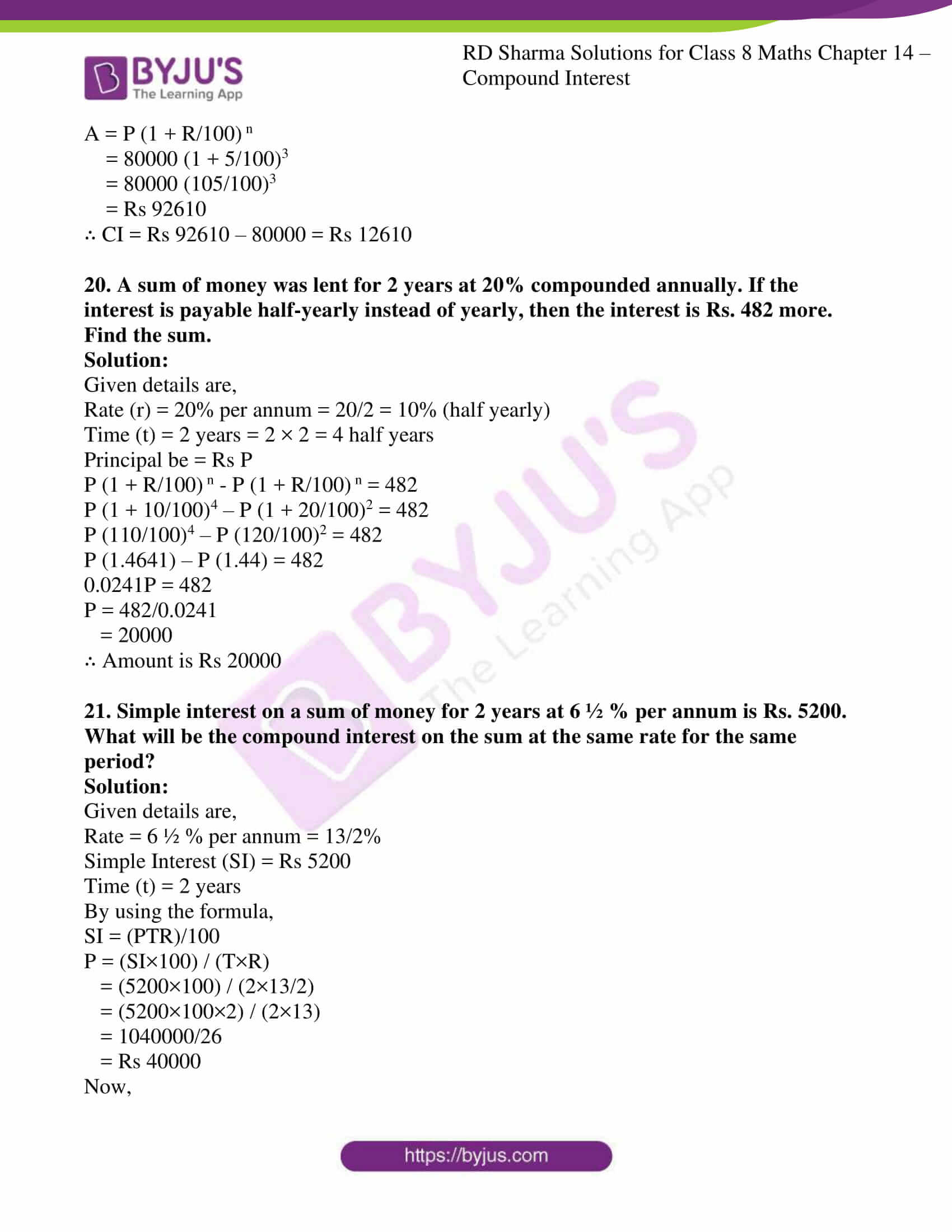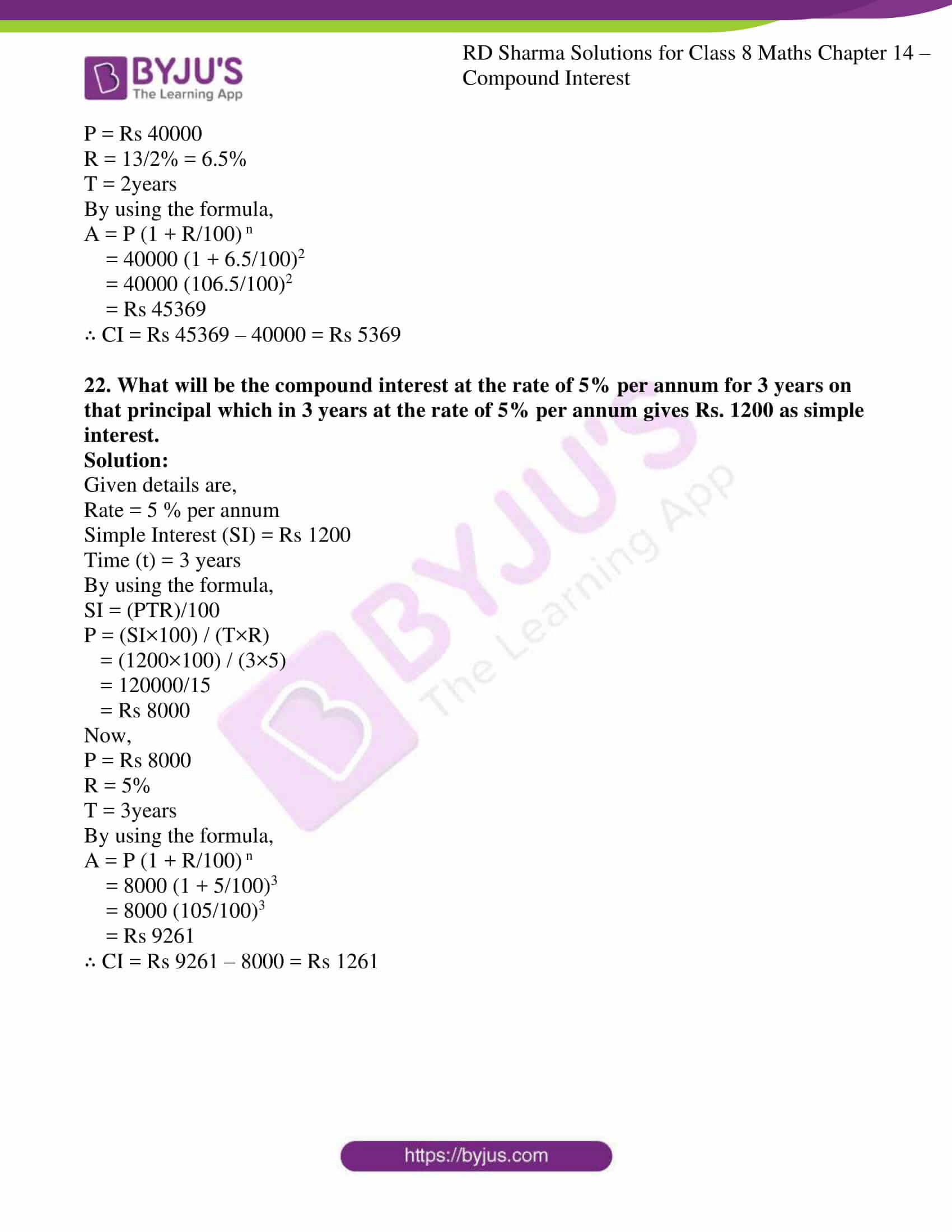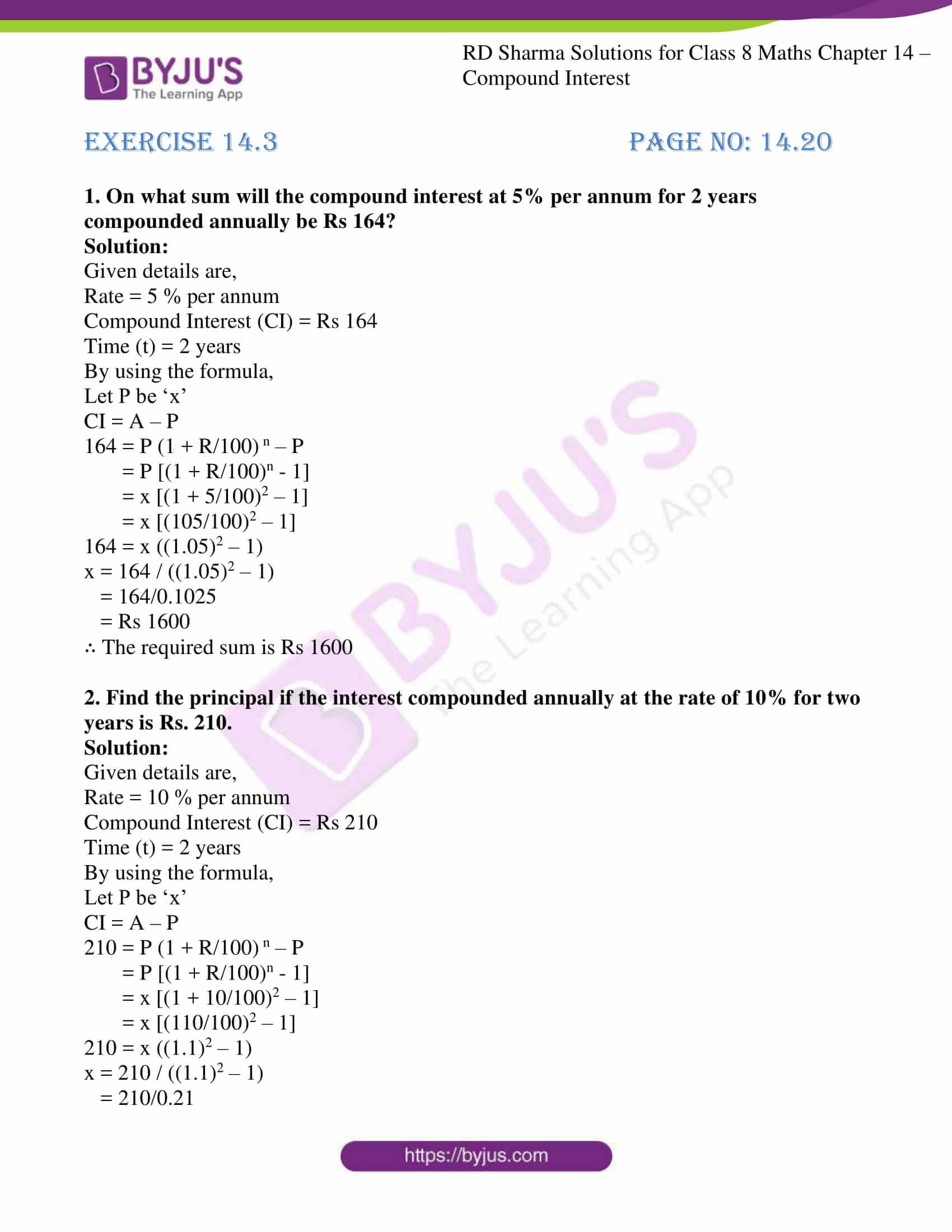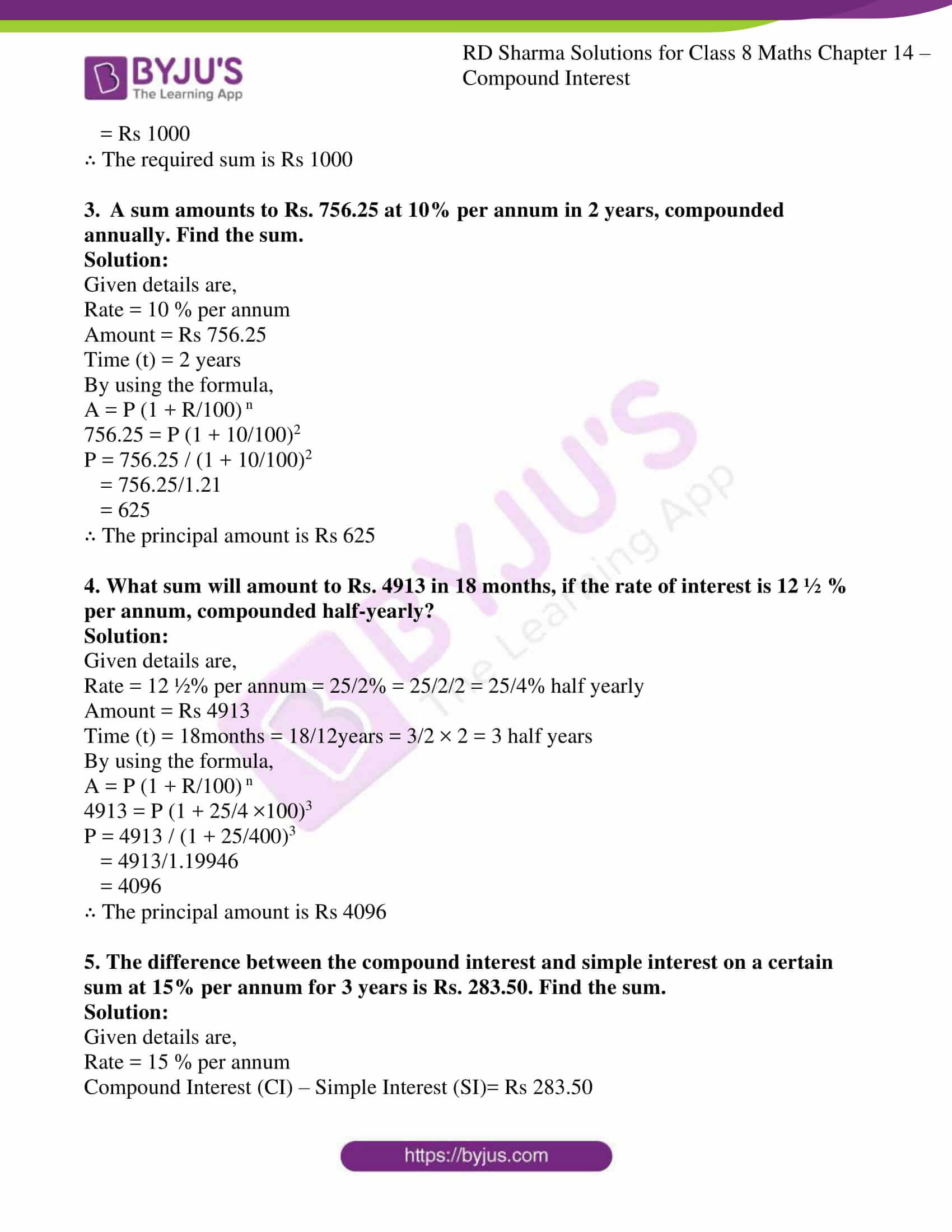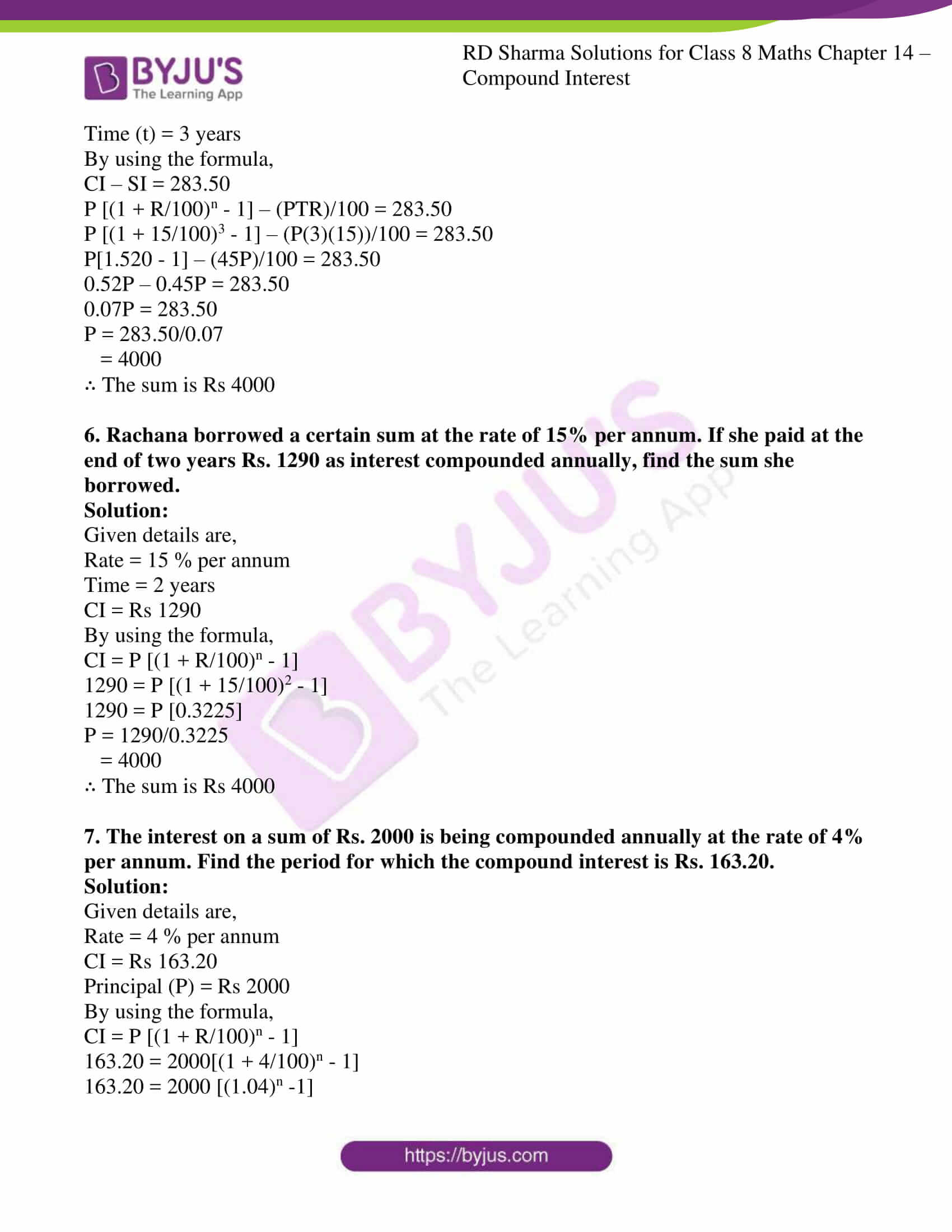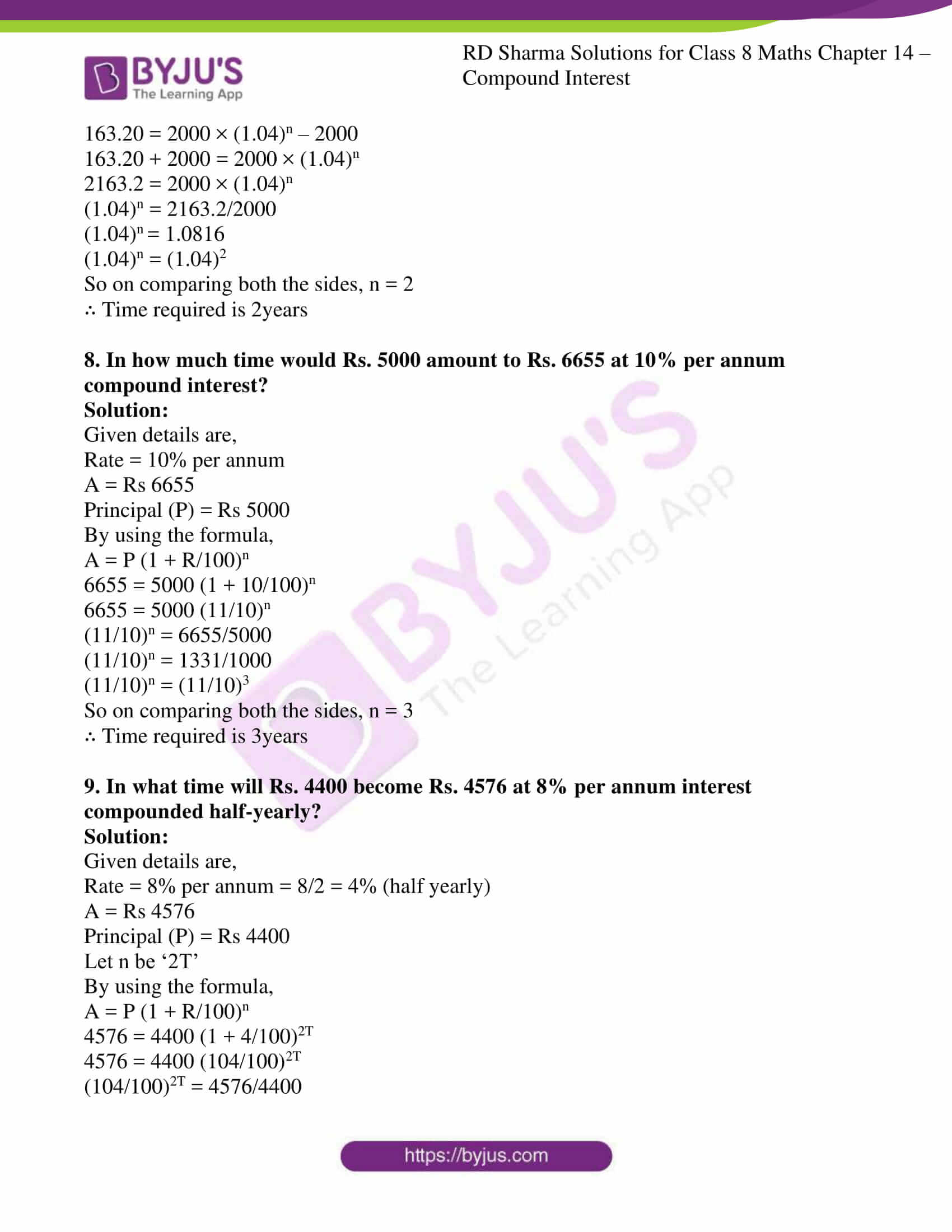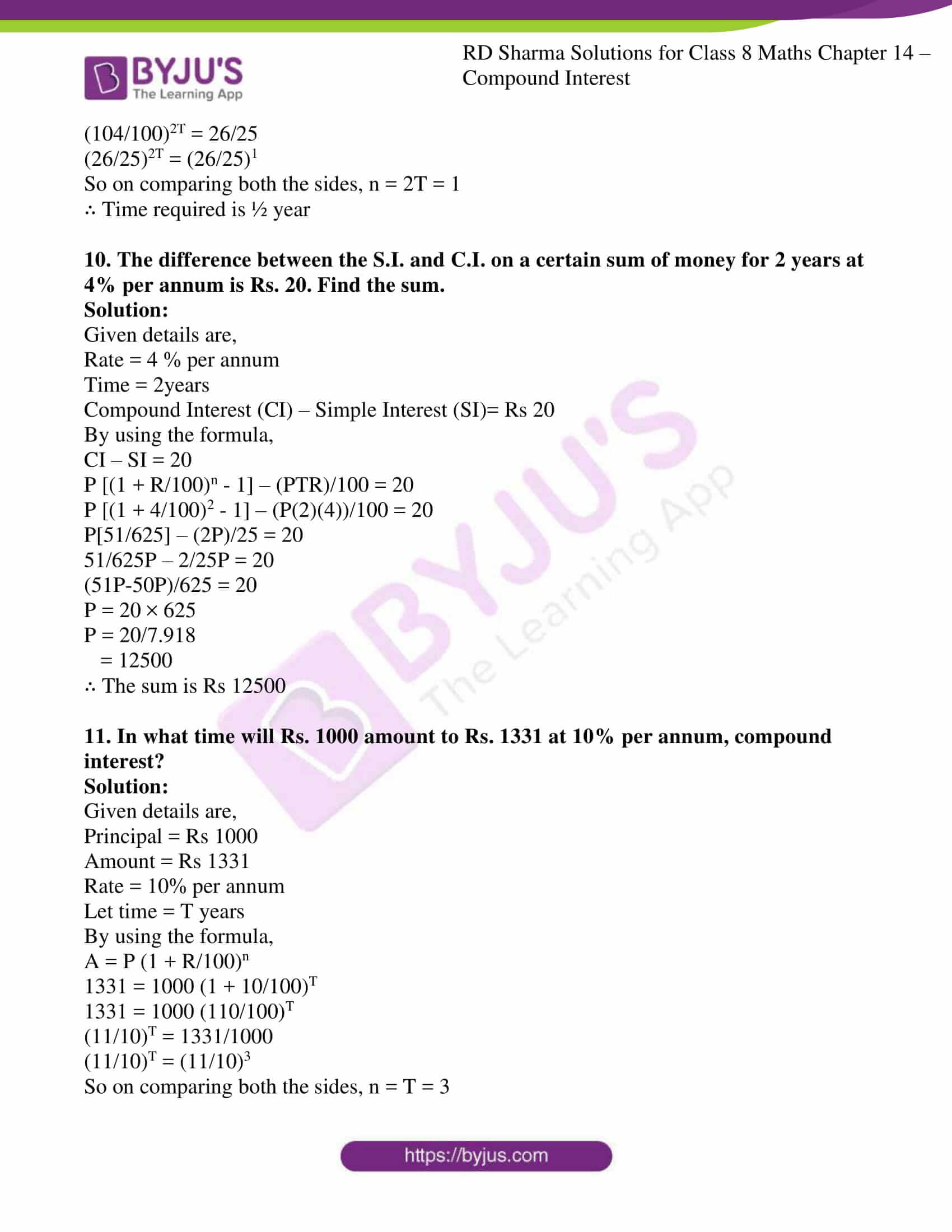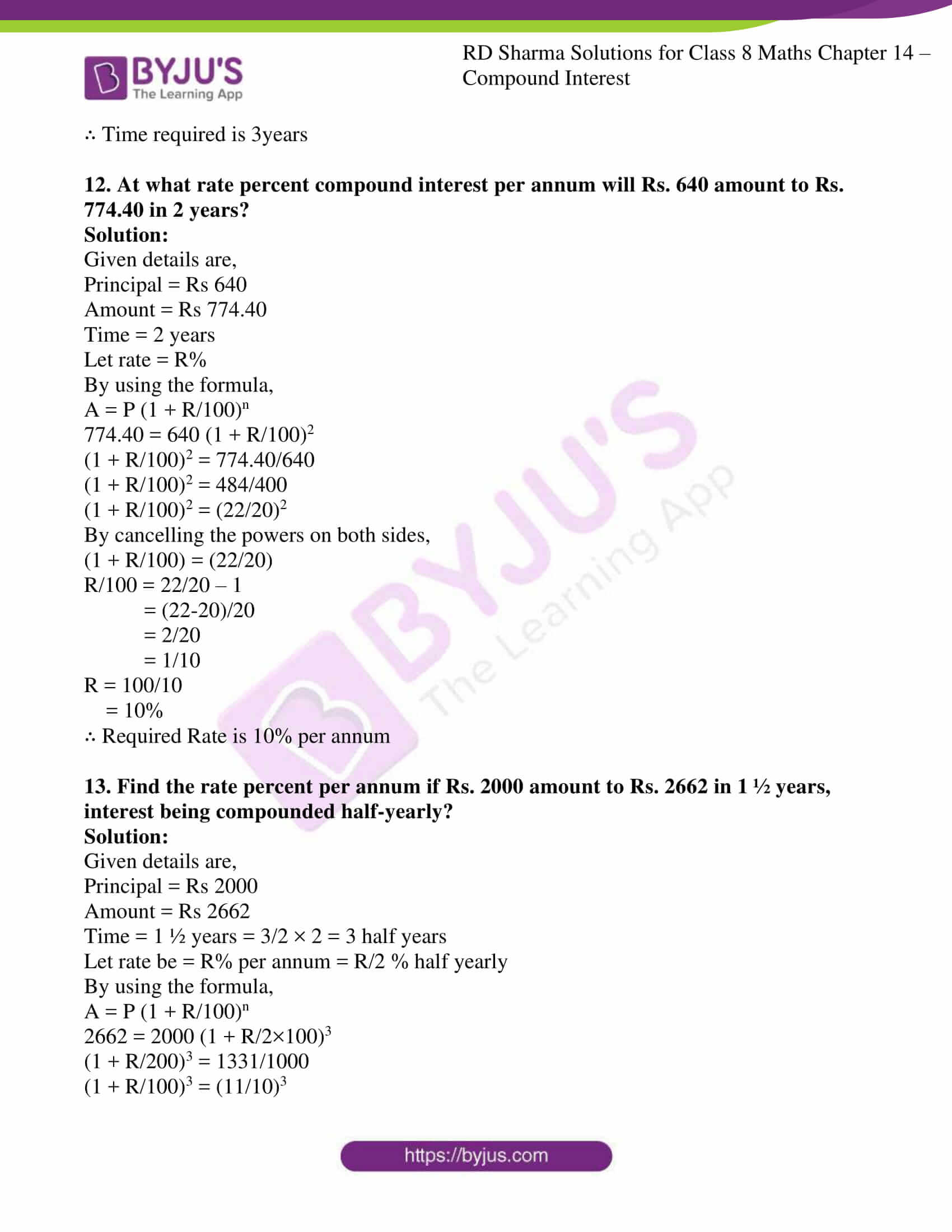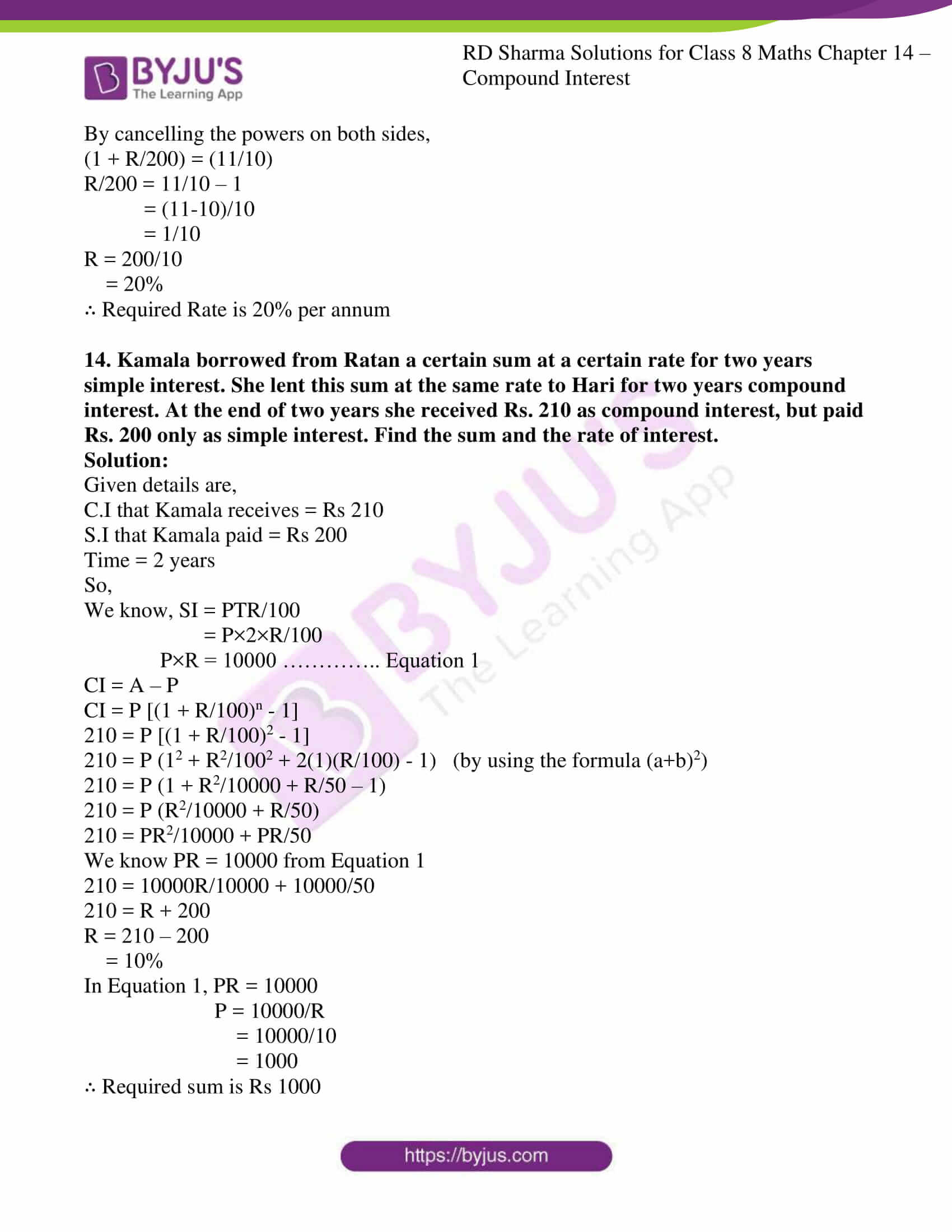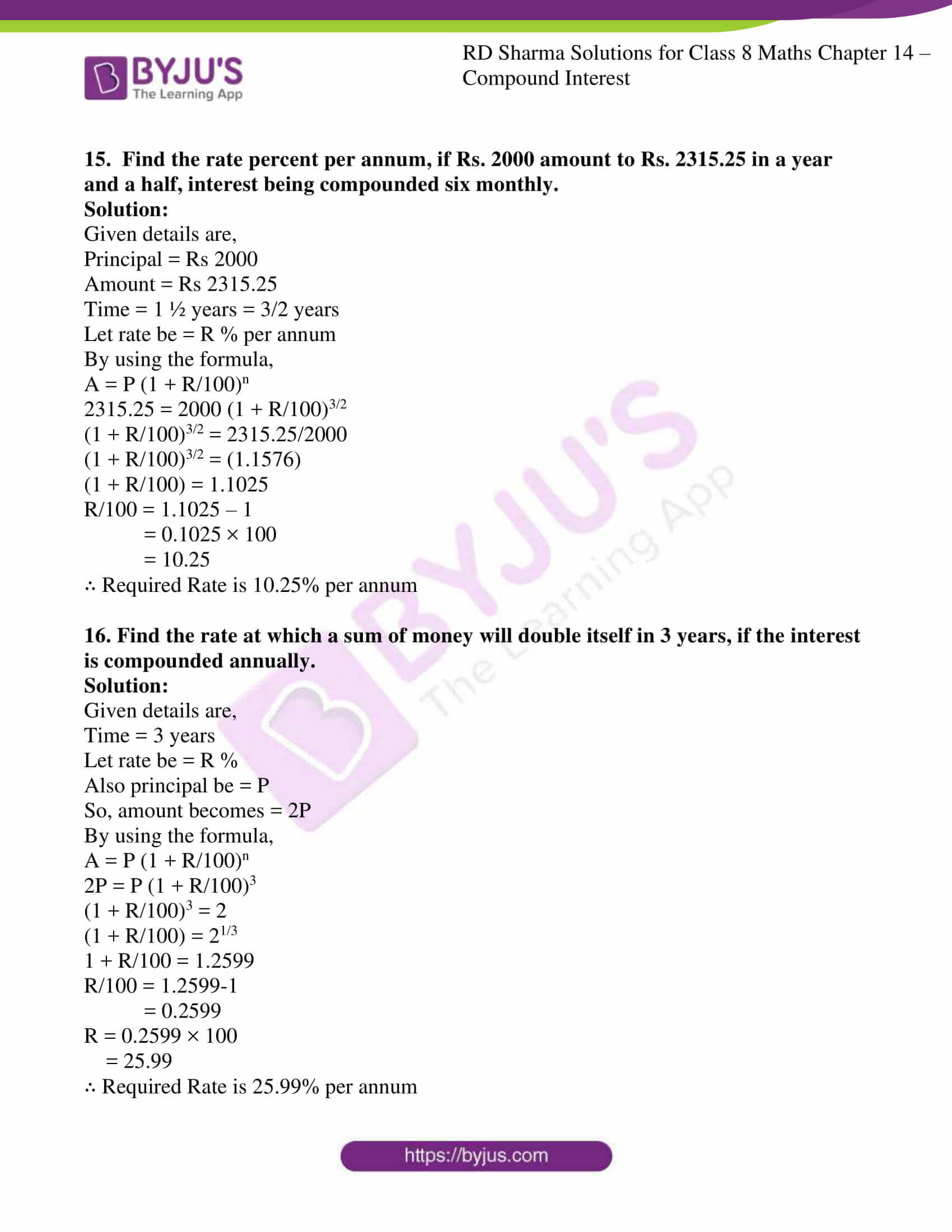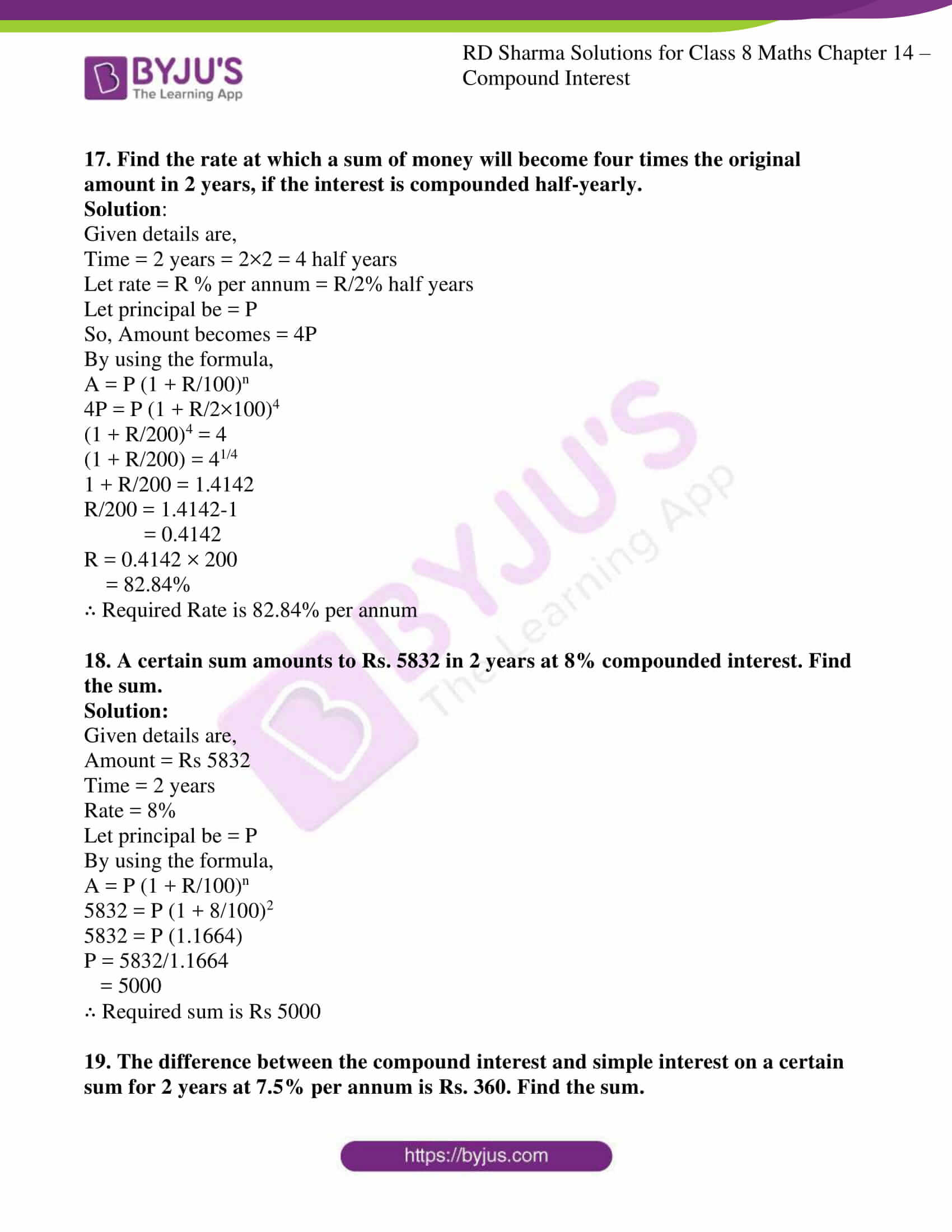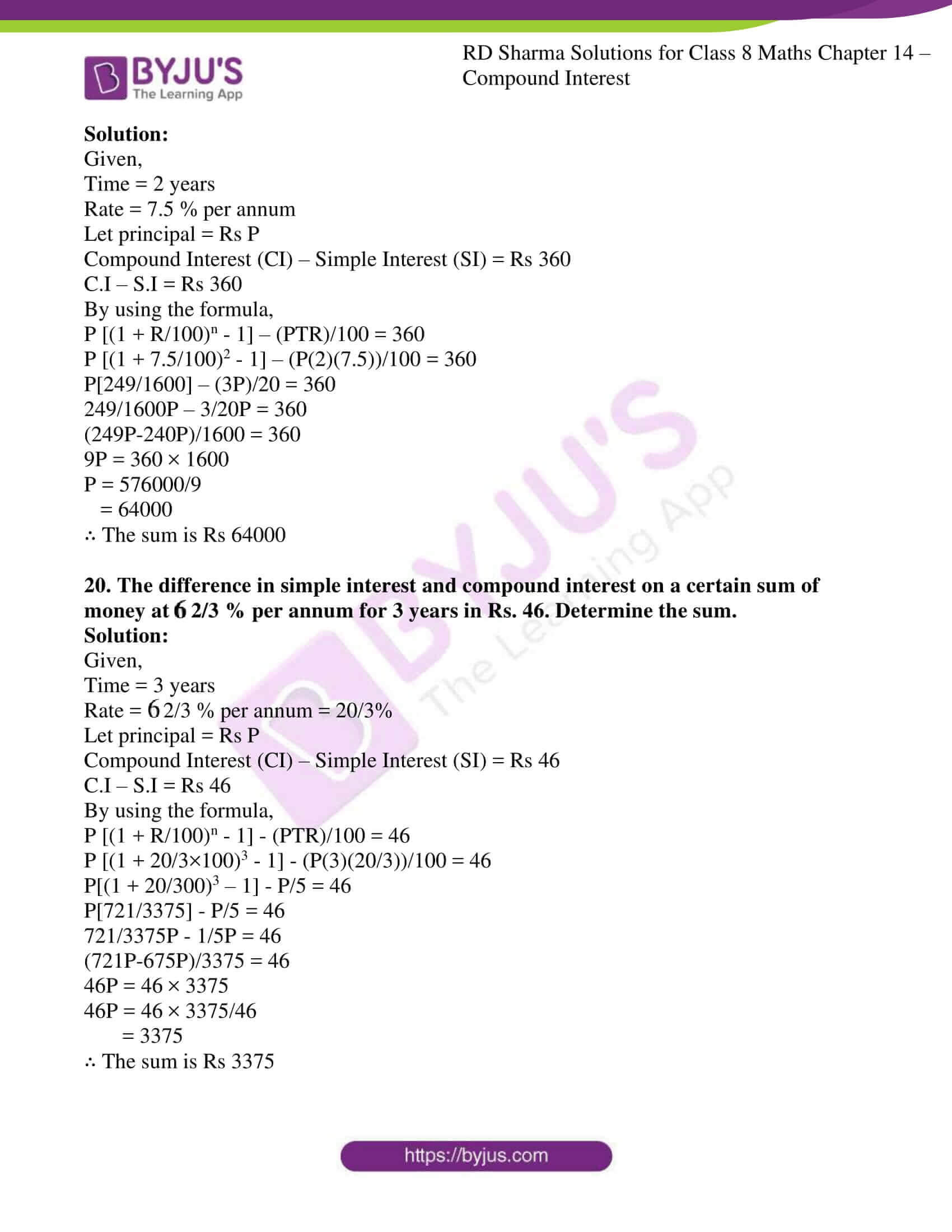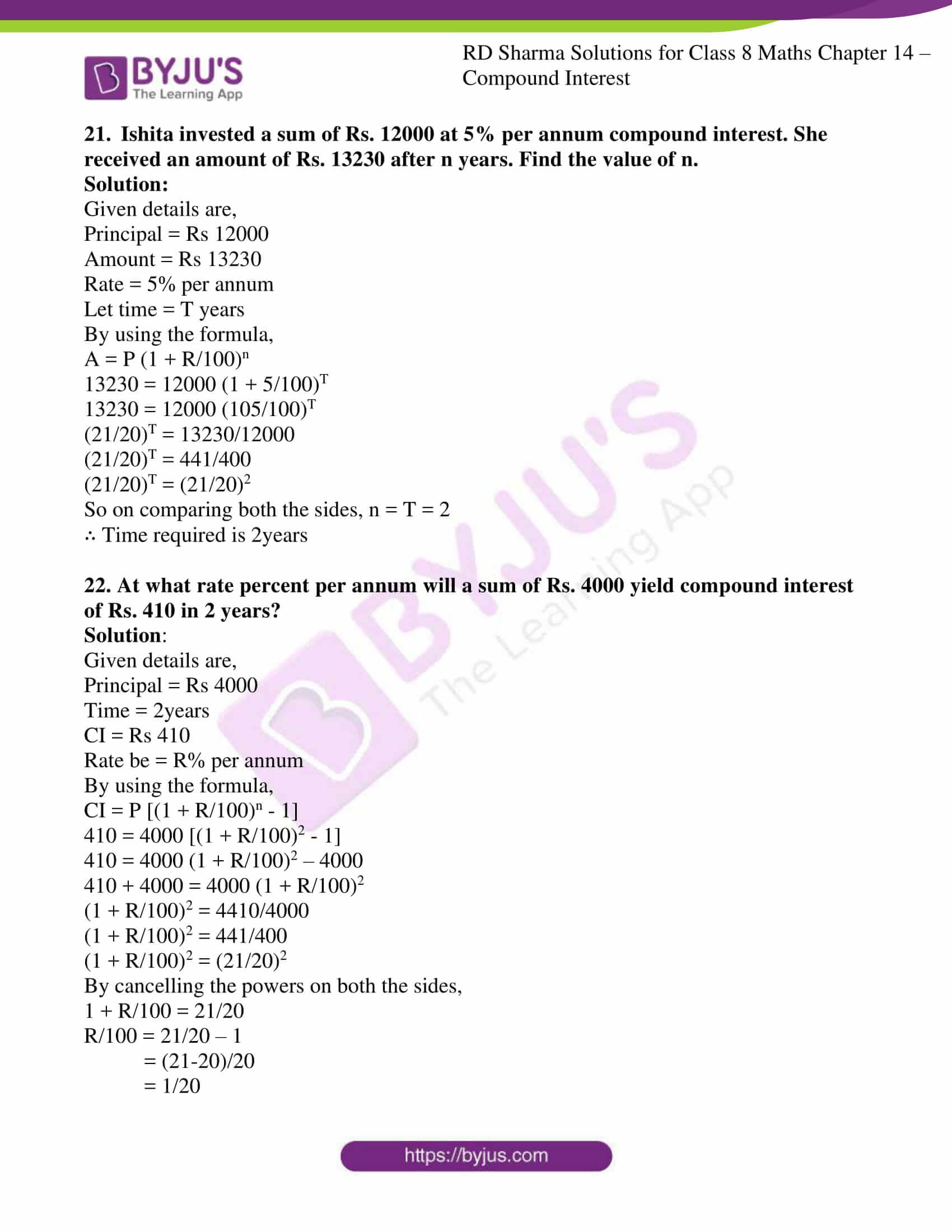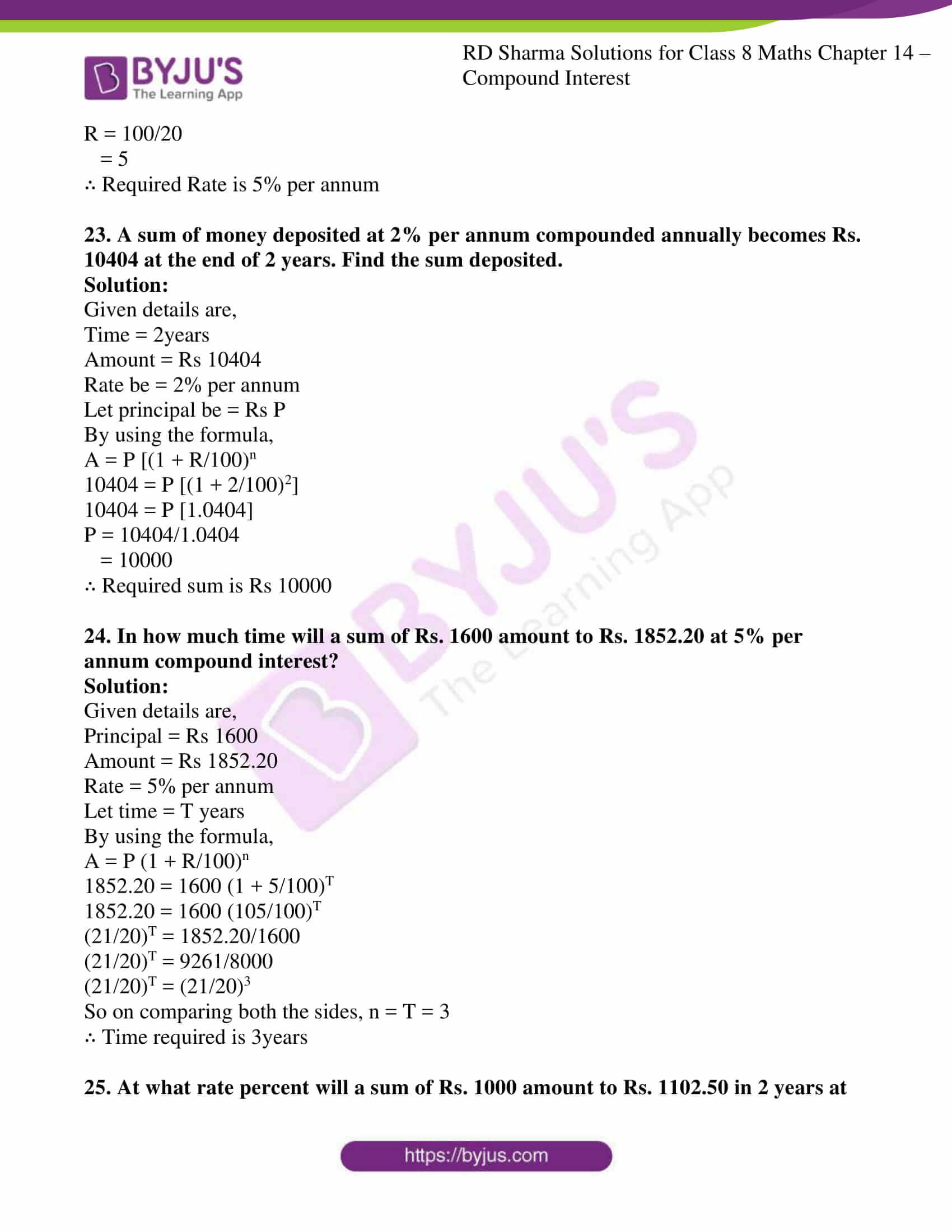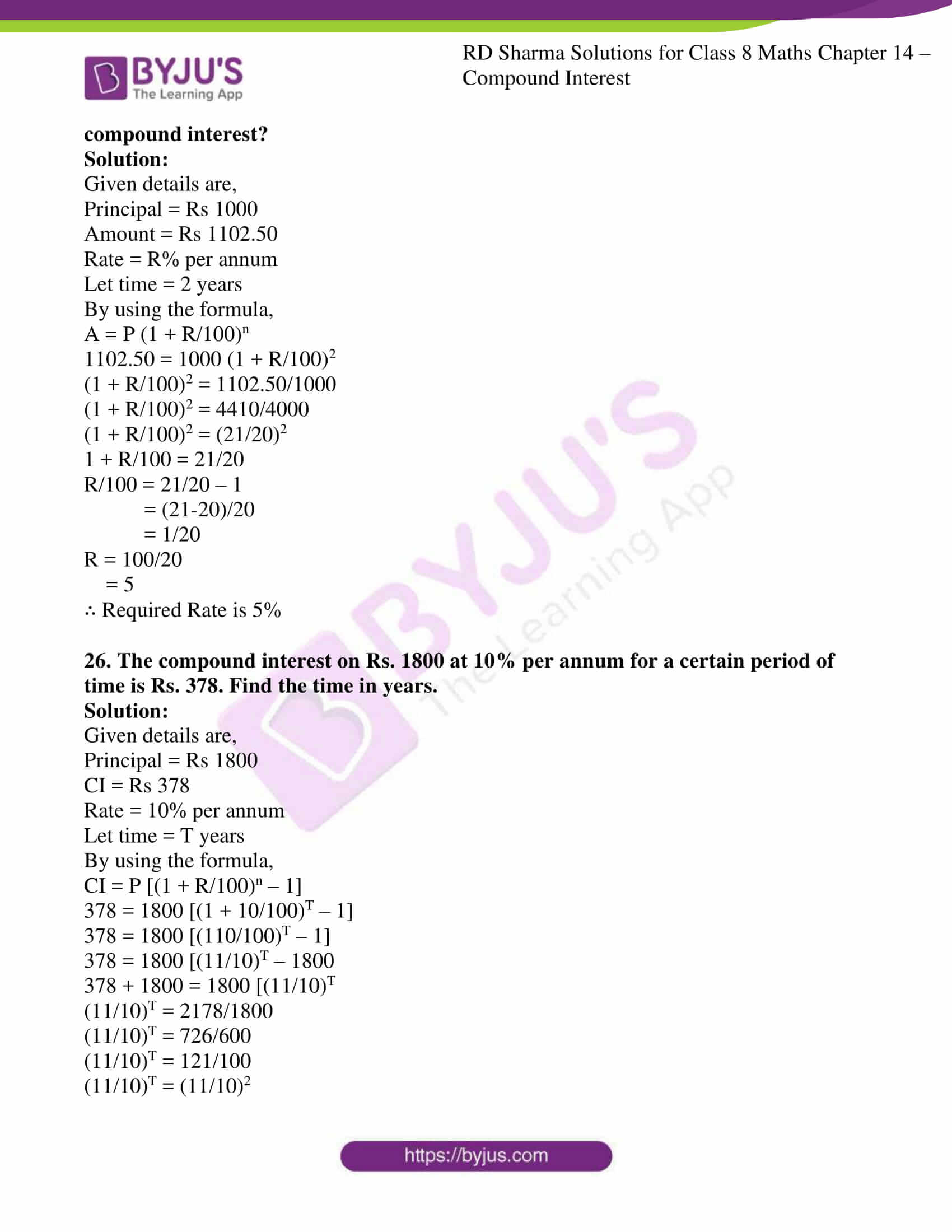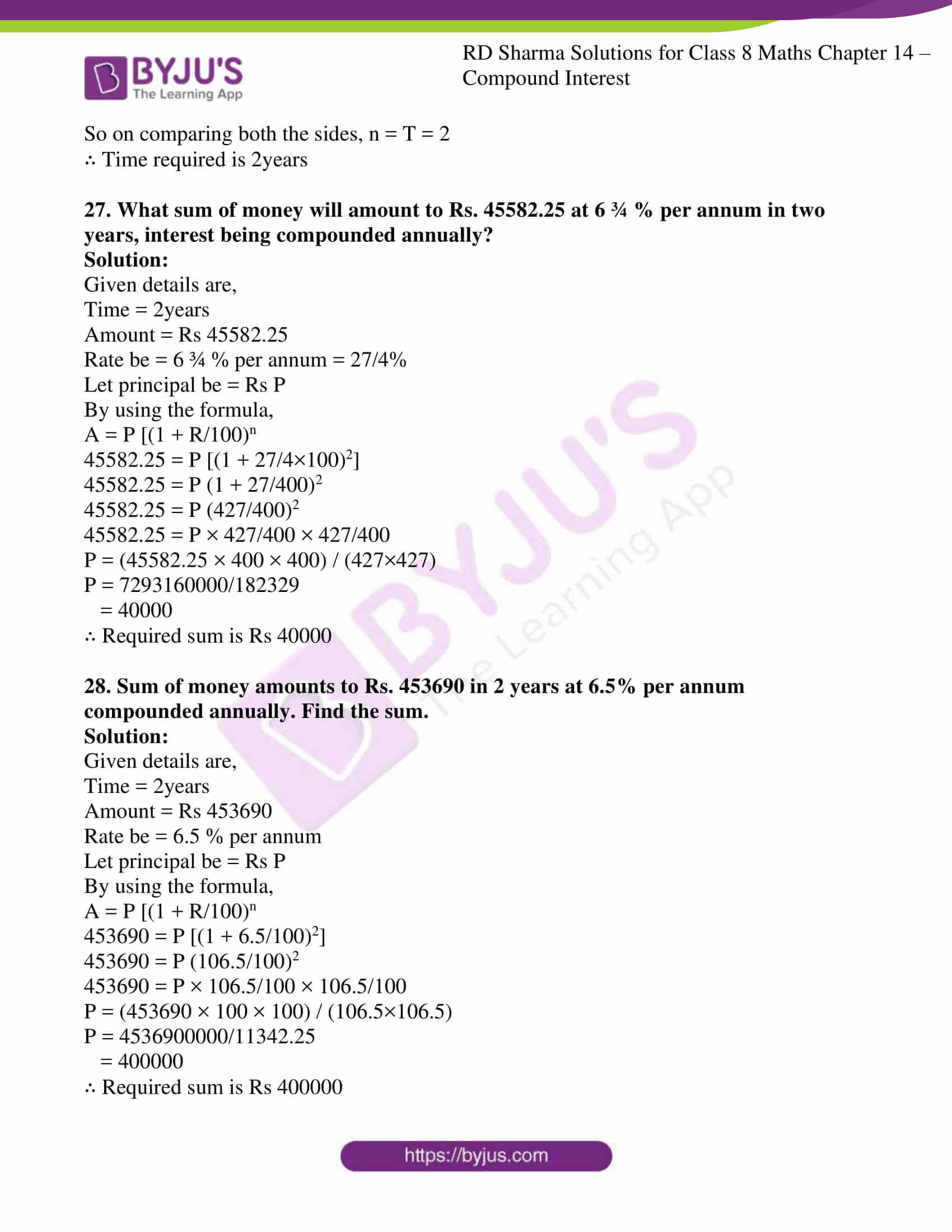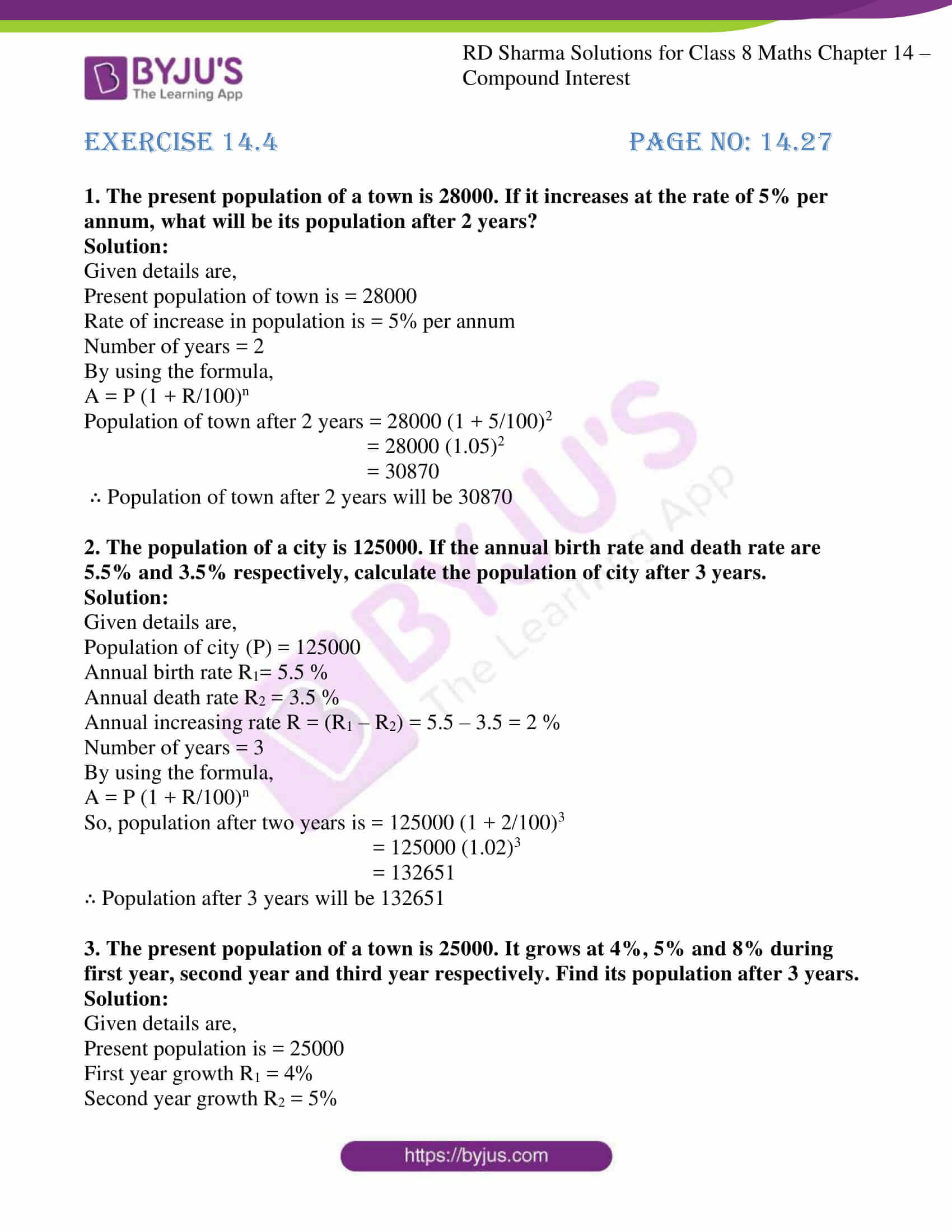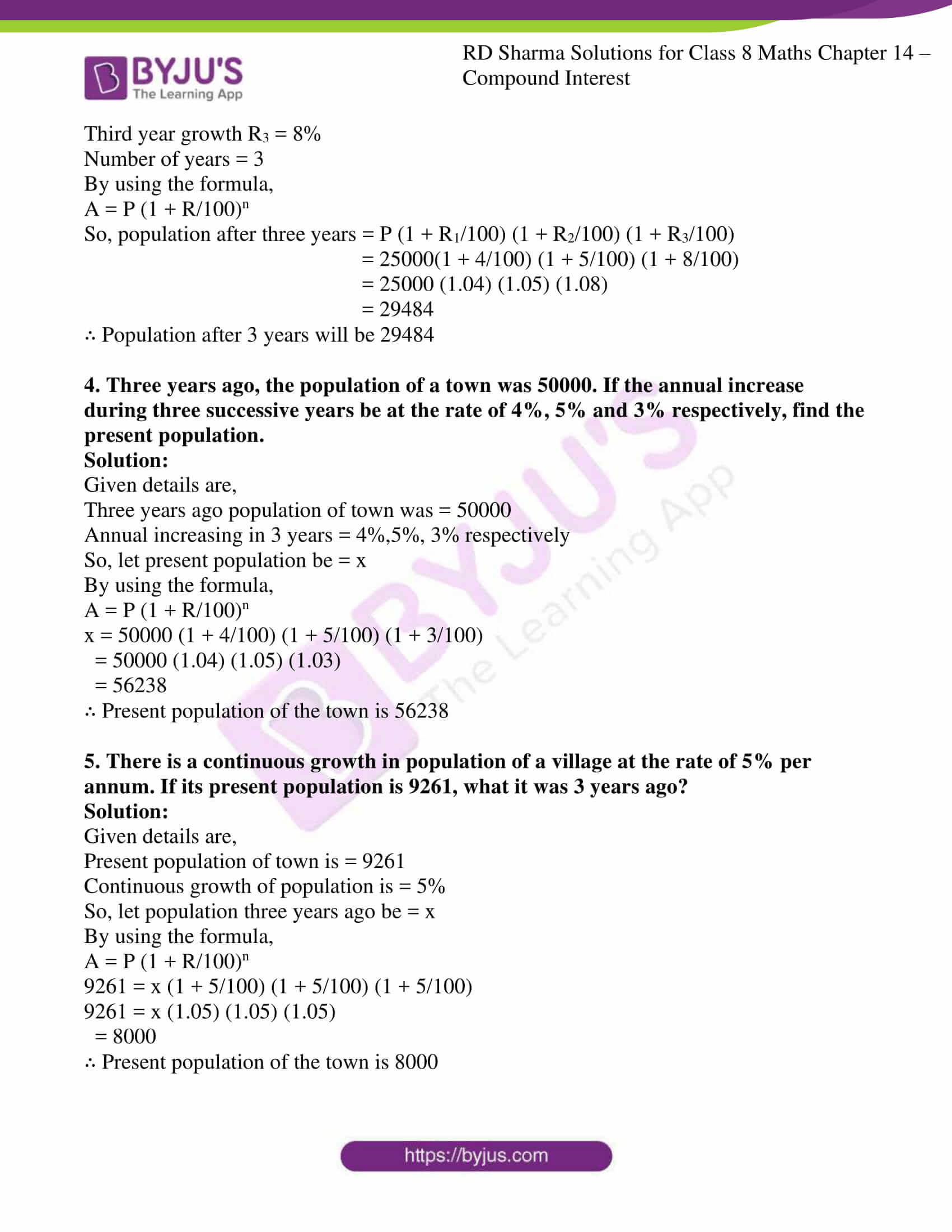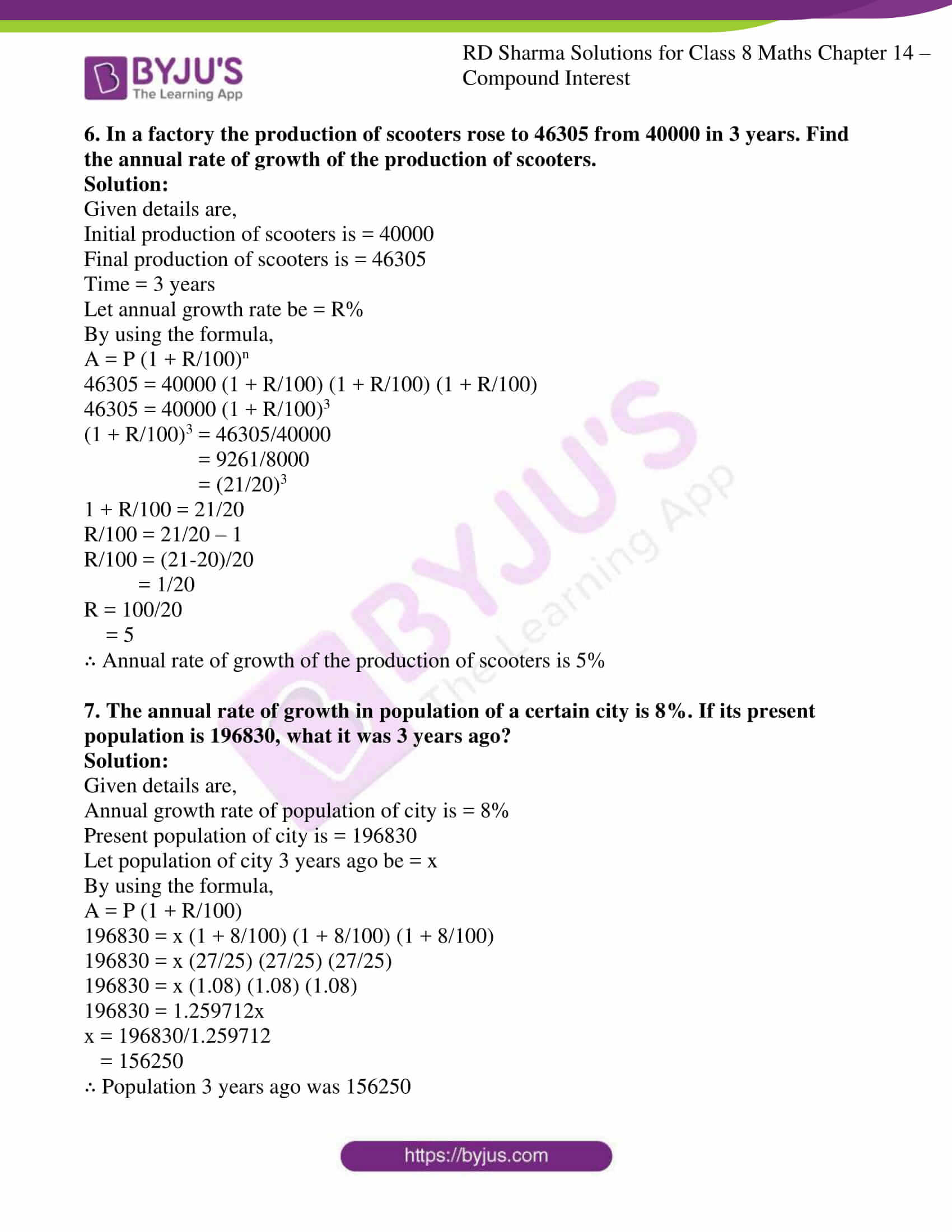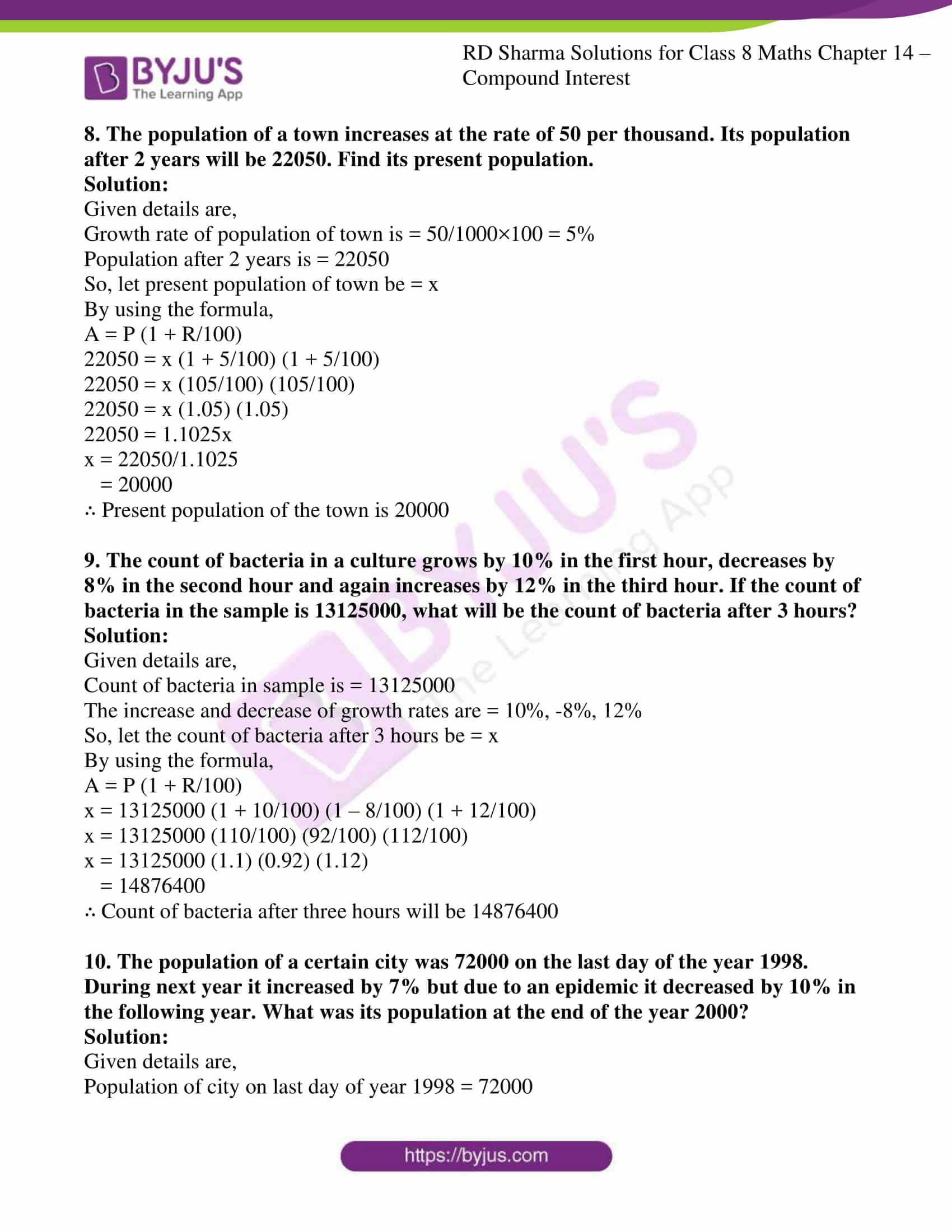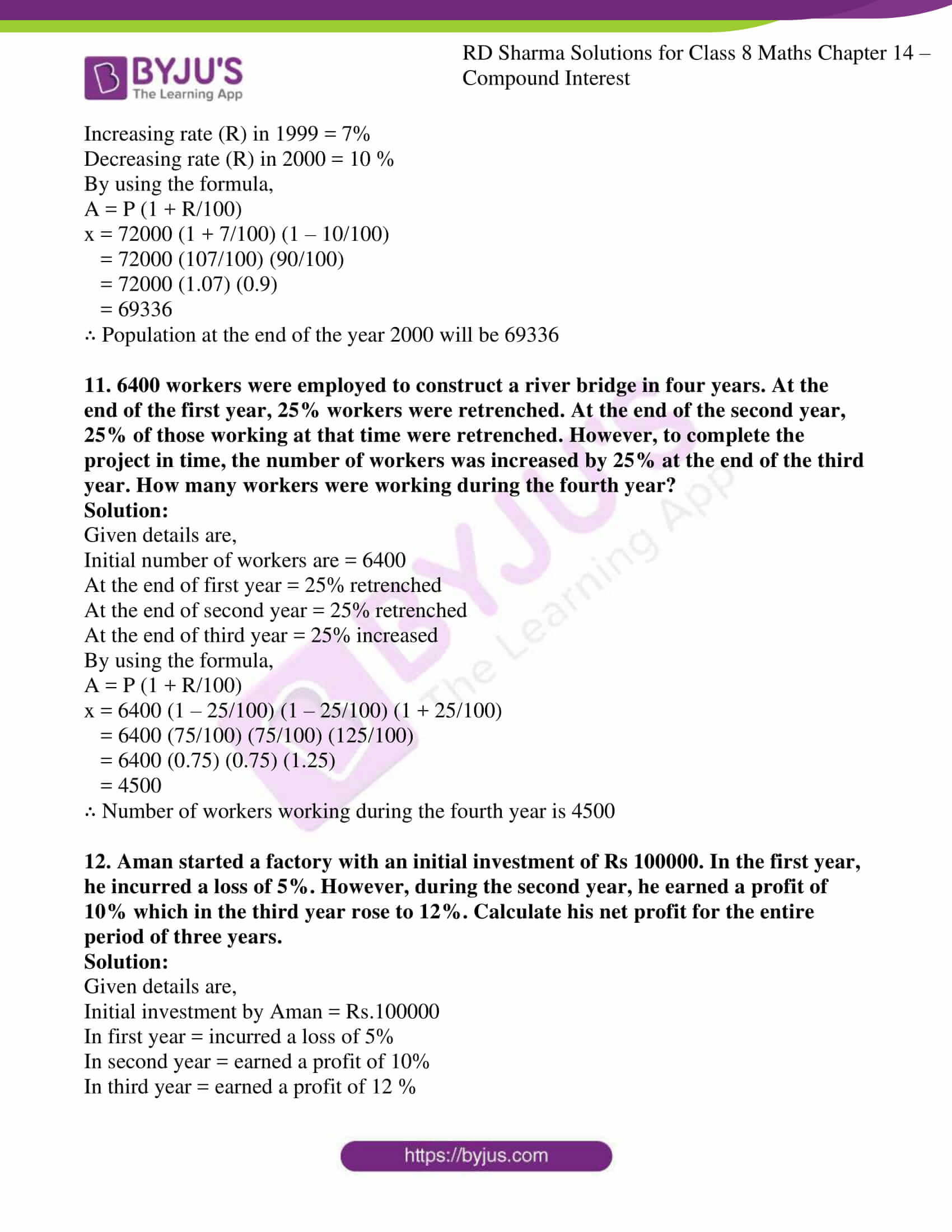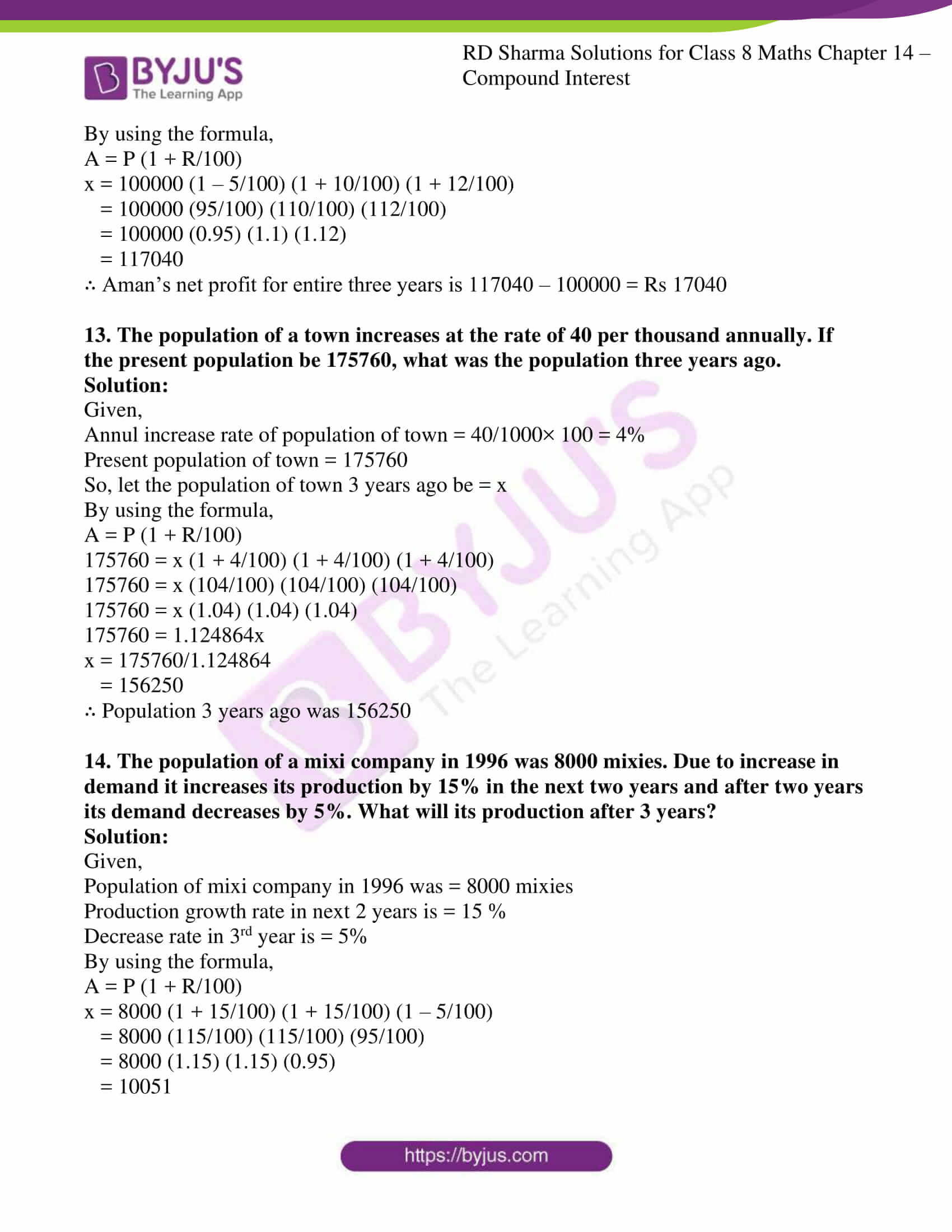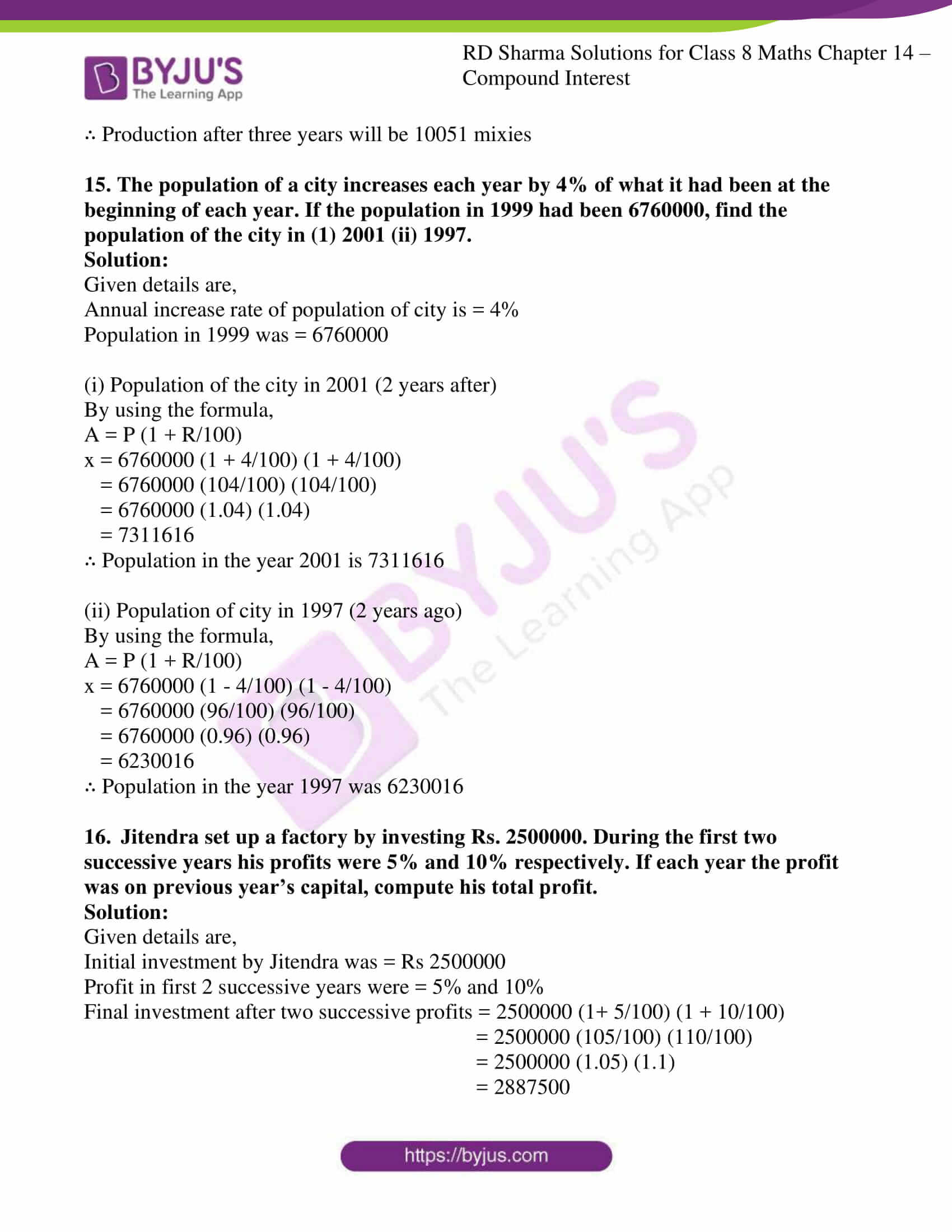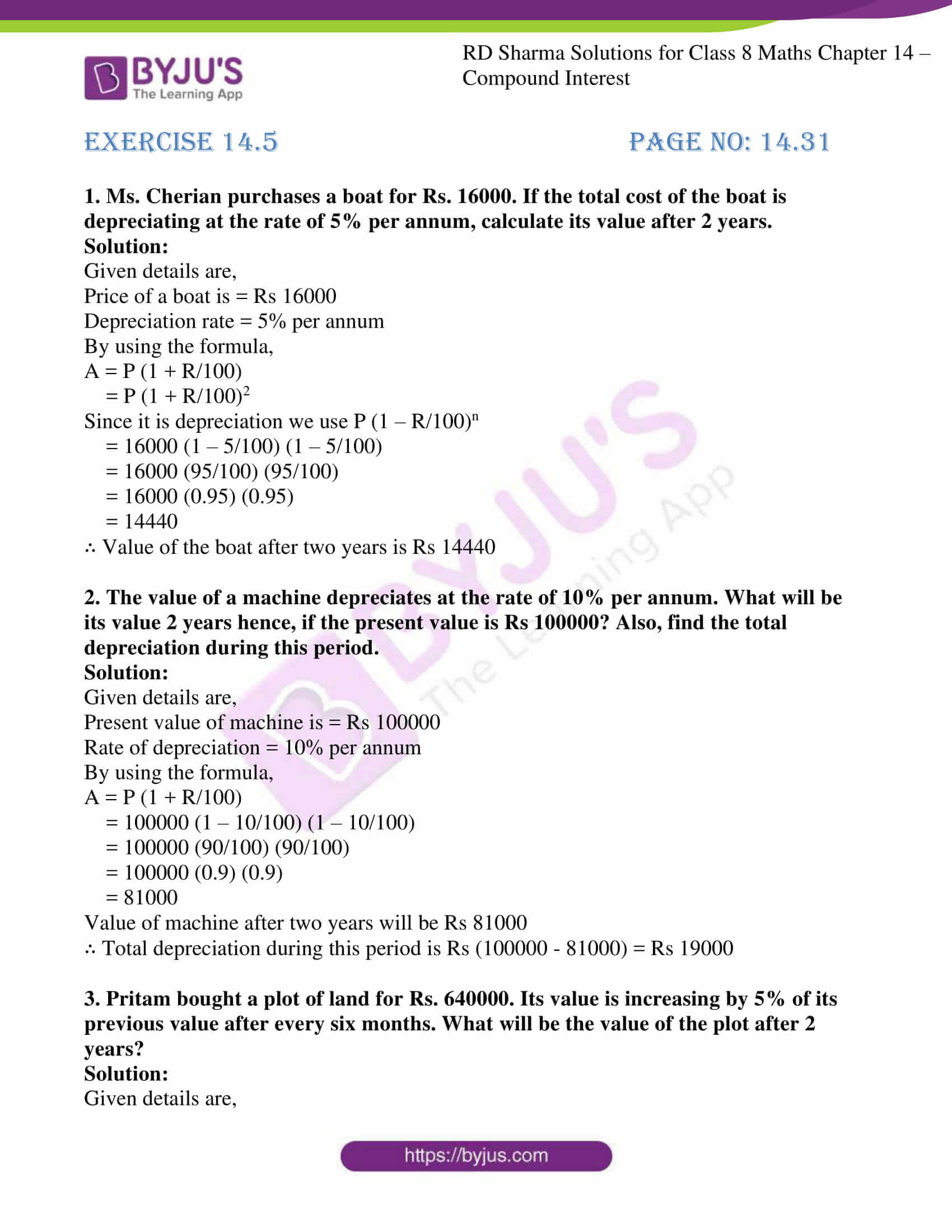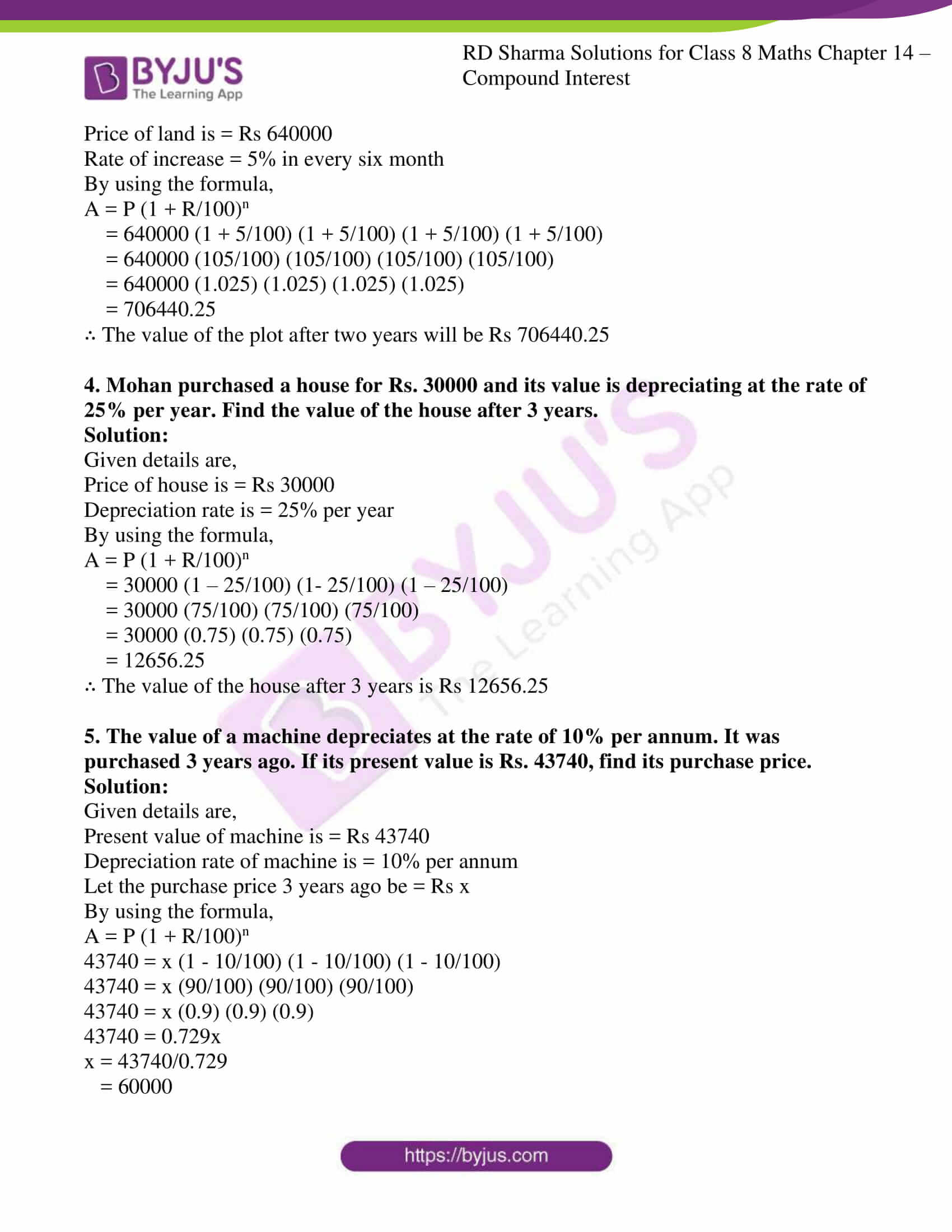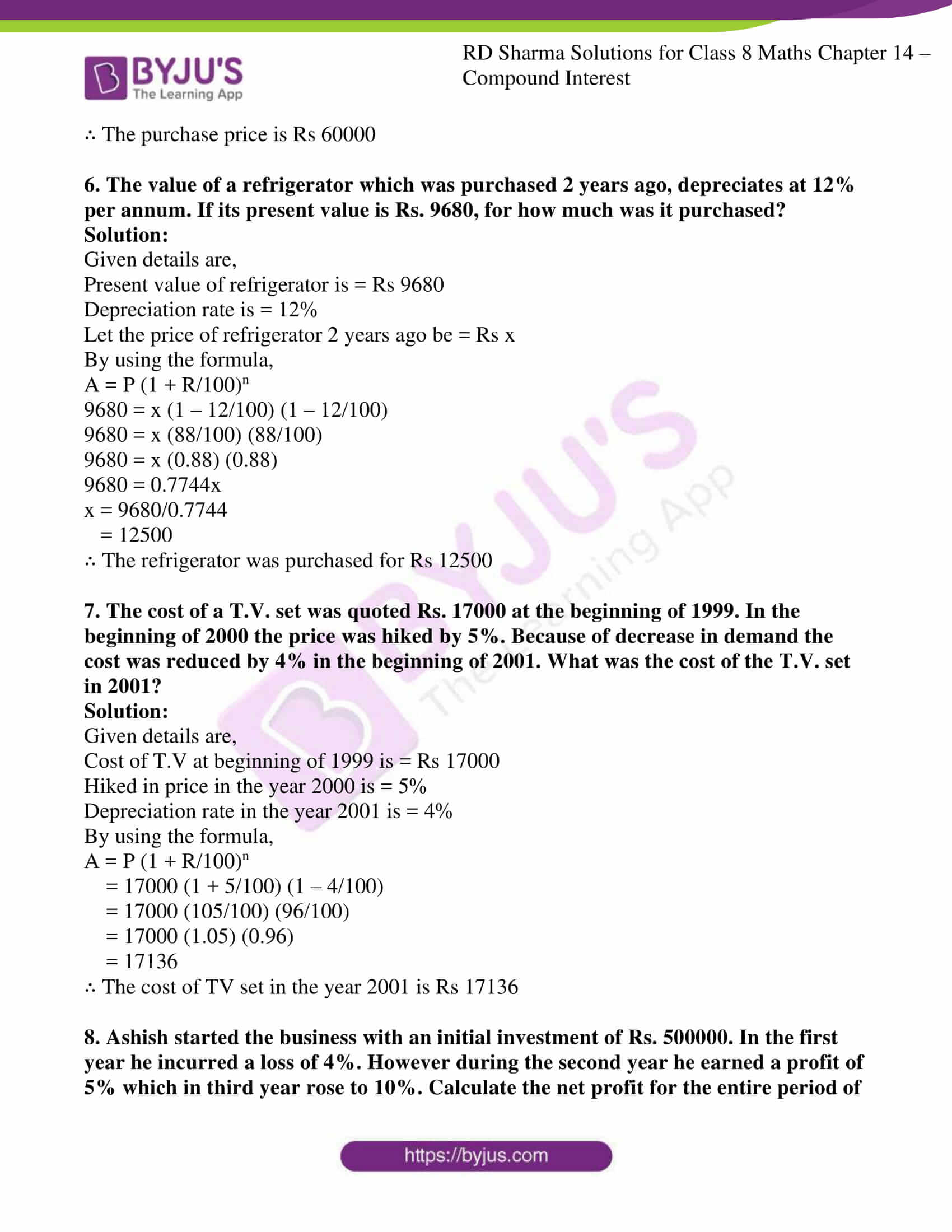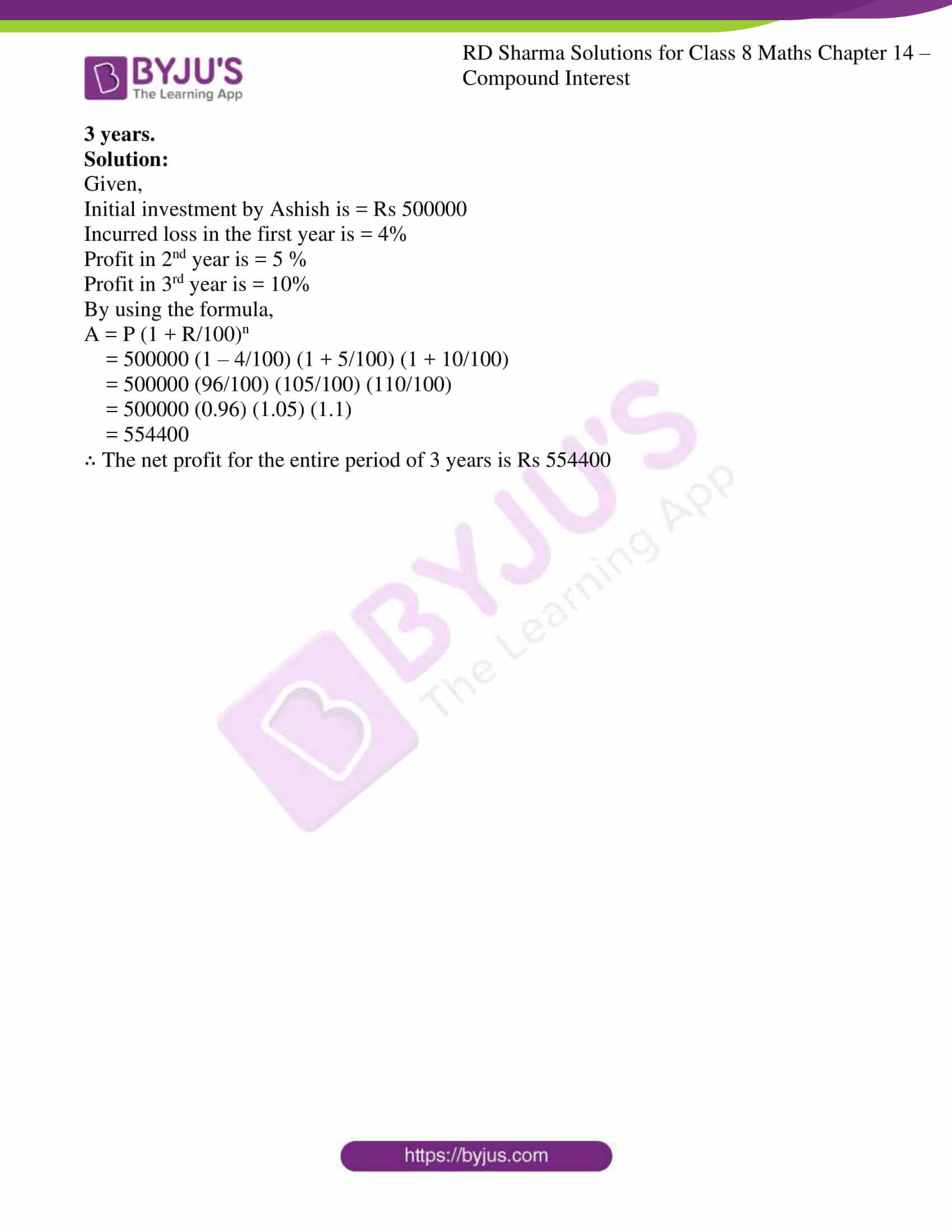### Access answers to Maths RD Sharma Solutions For Class 8 Chapter 14 Compound Interest

EXERCISE 14.1 PAGE NO: 14.4

1. Find the compound interest when principal = Rs 3000, rate = 5% per annum and time = 2 years.

Solution:

Given details are,

Principal (p) = Rs 3000

Rate (r) = 5%

Time = 2years

Interest for the first year = (3000×5×1)/100 = 150

Amount at the end of first year = Rs 3000 + 300 = Rs 3150

Principal interest for the second year = (3150×5×1)/100 = 157.5

Amount at the end of second year = Rs 3150 + 157.5 = Rs 3307.5

∴ Compound Interest = Rs 3307.5 – Rs 3000 = Rs 307.5

2. What will be the compound interest on Rs. 4000 in two years when rate of interest is 5% per annum?

Solution:

Given details are,

Principal (p) = Rs 4000

Rate (r) = 5%

Time = 2years

By using the formula,

A = P (1 + R/100) n

= 4000 (1 + 15/100)3

= 4000 (105/100)3

= Rs 4410

∴ Compound Interest = A – P = Rs 4410 – Rs 4000 = Rs 410

3. Rohit deposited Rs. 8000 with a finance company for 3 years at an interest of 15% per annum. What is the compound interest that Rohit gets after 3 years?

Solution:

Given details are,

Principal (p) = Rs 8000

Rate (r) = 15%

Time = 3years

By using the formula,

A = P (1 + R/100) n

= 8000 (1 + 15/100)3

= 8000 (115/100)3

= Rs 12167

∴ Compound Interest = A – P = Rs 12167 – Rs 8000 = Rs 4167

4. Find the compound interest on Rs. 1000 at the rate of 8% per annum for 1 ½ years when interest is compounded half yearly.

Solution:

Given details are,

Principal (p) = Rs 1000

Rate (r) = 8%

Time = 1 ½ years = 3/2 × 2 = 3 half years

By using the formula,

A = P (1 + R/200) 2n

= 1000 (1 + 8/200)3

= 1000 (208/200)3

= Rs 1124.86

∴ Compound Interest = A – P = Rs 1124.86 – Rs 1000 = Rs 124.86

5. Find the compound interest on Rs. 160000 for one year at the rate of 20% per annum, if the interest is compounded quarterly.

Solution:

Given details are,

Principal (p) = Rs 160000

Rate (r) = 20% = 20/4 = 5% (for quarter year)

Time = 1year = 1 × 4 = 4 quarters

By using the formula,

A = P (1 + R/100) n

= 160000 (1 + 5/100)4

= 160000 (105/100)4

= Rs 194481

∴ Compound Interest = A – P = Rs 194481 – Rs 160000 = Rs 34481

6. Swati took a loan of Rs. 16000 against her insurance policy at the rate of 12 ½ % per annum. Calculate the total compound interest payable by Swati after 3 years.

Solution:

Given details are,

Principal (p) = Rs 16000

Rate (r) = 12 ½ % = 12.5%

Time = 3years

By using the formula,

A = P (1 + R/100) n

= 16000 (1 + 12.5/100)3

= 16000 (112.5/100)3

= Rs 22781.25

∴ Compound Interest = A – P = Rs 22781.25 – Rs 16000 = Rs 6781.25

7. Roma borrowed Rs. 64000 from a bank for 1 ½ years at the rate of 10% per annum. Compare the total compound interest payable by Roma after 1 ½ years, if the interest is compounded half-yearly.

Solution:

Given details are,

Principal (p) = Rs 64000

Rate (r) = 10 % = 10/2 % (for half a year)

Time = 1 ½ years = 3/2 × 2 = 3 (half year)

By using the formula,

A = P (1 + R/100) n

= 64000 (1 + 10/2×100)3

= 64000 (210/200)3

= Rs 74088

∴ Compound Interest = A – P = Rs 74088 – Rs 64000 = Rs 10088

8. Mewa lal borrowed Rs. 20000 from his friend Rooplal at 18% per annum simple interest. He lent it to Rampal at the same rate but compounded annually. Find his gain after 2 years.

Solution:

Given details are,

Principal (p) = Rs 20000

Rate (r) = 18 %

Time = 2 years

By using the formula,

Interest amount Mewa lal has to pay,

By using the formula,

Simple interest = P×T×R/100

= (20000×18×2)/100 = 7200

Interest amount Rampal has to pay to Mewa lal,

By using the formula,

A = P (1 + R/100) n

= 20000 (1 + 18/100)2

= 20000 (118/100)2

= Rs 27848 – 20000 (principal amount)

= Rs 7848

∴ Mewa lal gain = Rs (7848 – 7200) = Rs 648

9. Find the compound interest on Rs. 8000 for 9 months at 20% per annum compounded quarterly.

Solution:

Given details are,

Principal (p) = Rs 8000

Rate (r) = 20 % = 20/4 = 5% (for quarterly)

Time = 9 months = 9/3 = 3 (for quarter year)

By using the formula,

A = P (1 + R/100) n

= 8000 (1 + 5/100)3

= 8000 (105/100)3

= Rs 9261

∴ Compound Interest = A – P = Rs 9261 – Rs 8000 = Rs 1261

10. Find the compound interest at the rate of 10% per annum for two years on that principal which in two years at the rate of 10% per annum given Rs. 200 as simple interest.

Solution:

Given details are,

Simple interest (SI) = Rs 200

Rate (r) = 10 %

Time = 2 years

So, by using the formula,

Simple interest = P×T×R/100

P = (SI × 100)/ T×R

= (200 × 100) / 2 × 10

= 20000/20

= Rs 1000

Now,

Rate of compound interest = 10%

Time = 2years

By using the formula,

A = P (1 + R/100) n

= 1000 (1 + 10/100)2

= 1000 (110/100)2

= Rs 1210

∴ Compound Interest = A – P = Rs 1210 – Rs 1000 = Rs 210

11. Find the compound interest on Rs. 64000 for 1 year at the rate of 10% per annum compounded quarterly.

Solution:

Given details are,

Principal (p) = Rs 64000

Rate (r) = 10 % = 10/4 % (for quarterly)

Time = 1year = 1× 4 = 4 (for quarter in a year)

By using the formula,

A = P (1 + R/100) n

= 64000 (1 + 10/4×100)4

= 64000 (410/400)4

= Rs 70644.03

∴ Compound Interest = A – P = Rs 70644.03 – Rs 64000 = Rs 6644.03

12. Ramesh deposited Rs. 7500 in a bank which pays him 12% interest per annum compounded quarterly. What is the amount which he receives after 9 months.

Solution:

Given details are,

Principal (p) = Rs 7500

Rate (r) = 12 % = 12/4 = 3 % (for quarterly)

Time = 9 months = 9/12years = 9/12 × 4 = 3 (for quarter in a year)

By using the formula,

A = P (1 + R/100) n

= 7500 (1 + 3/100)3

= 7500 (103/100)3

= Rs 8195.45

∴ Required amount is Rs 8195.45

13. Anil borrowed a sum of Rs. 9600 to install a hand pump in his dairy. If the rate of interest is 5 ½ % per annum compounded annually, determine the compound interest which Anil will have to pay after 3 years.

Solution:

Given details are,

Principal (p) = Rs 9600

Rate (r) = 5 ½ % = 11/2 %

Time = 3years

By using the formula,

A = P (1 + R/100) n

= 9600 (1 + 11/2×100)3

= 9600 (211/200)3

= Rs 11272.71

∴ Compound Interest = A – P = Rs 11272.71 – Rs 9600 = Rs 1672.71

14. Surabhi borrowed a sum of Rs. 12000 from a finance company to purchase a refrigerator. If the rate of interest is 5% per annum compounded annually, calculate the compound interest that Surabhi has to pay to the company after 3 years.

Solution:

Given details are,

Principal (p) = Rs 12000

Rate (r) = 5 %

Time = 3years

By using the formula,

A = P (1 + R/100) n

= 12000 (1 + 5/100)3

= 12000 (105/100)3

= Rs 13891.5

∴ Compound Interest = A – P = Rs 13891.5 – Rs 12000 = Rs 1891.5

15. Daljit received a sum of Rs. 40000 as a loan from a finance company. If the rate of interest is 7% per annum compounded annually, calculate the compound interest that Daljit pays after 2 years.

Solution:

Given details are,

Principal (p) = Rs 40000

Rate (r) = 7%

Time = 2years

By using the formula,

A = P (1 + R/100) n

= 40000 (1 + 7/100)2

= 40000 (107/100)2

= Rs 45796

∴ Compound Interest = A – P = Rs 45796 – Rs 40000 = Rs 5796

EXERCISE 14.2 PAGE NO: 14.14

1. Compute the amount and the compound interest in each of the following by using the formulae when :
(i) Principal = Rs 3000, Rate = 5%, Time = 2 years
(ii) Principal = Rs 3000, Rate = 18%, Time = 2 years
(iii) Principal = Rs 5000, Rate = 10 paise per rupee per annum, Time = 2 years
(iv) Principal = Rs 2000, Rate = 4 paise per rupee per annum, Time = 3 years
(v) Principal = Rs 12800, Rate = 7 ½ %, Time = 3 years
(vi) Principal = Rs 10000, Rate = 20% per annum compounded half-yearly, Time = 2 years
(vii) Principal = Rs 160000, Rate = 10 paise per rupee per annum compounded half yearly, Time = 2 years.

Solution:

By using the formula,

A = P (1 + R/100) n

Let us solve

(i) Given, P = Rs 3000, rate = 5%, time = 2years

A = P (1 + R/100) n

= 3000 (1 + 5/100)2

= 3000 (105/100)2

= Rs 3307.5

Compound interest (CI) = A-P = Rs 3307.5 – 3000 = Rs 307.5

(ii) Given, P = Rs 3000, rate = 18%, time = 2years

A = P (1 + R/100) n

= 3000 (1 + 18/100)2

= 3000 (118/100)2

= Rs 4177.2

Compound interest (CI) = A-P = Rs 4177.2 – 3000 = Rs 1177.2

(iii) Given, P = Rs 5000, rate = 10%, time = 2years

A = P (1 + R/100) n

= 5000 (1 + 10/100)2

= 5000 (110/100)2

= Rs 6050

Compound interest (CI) = A-P = Rs 6050 – 5000 = Rs 1050

(iv) Given, P = Rs 2000, rate = 4%, time = 3years

A = P (1 + R/100) n

= 2000 (1 + 4/100)3

= 2000 (104/100)3

= Rs 2249.72

Compound interest (CI) = A-P = Rs 2249.72 – 2000 = Rs 249.72

(v) Given, P = Rs 12800, rate = 7 ½ % = 15/2% = 7.5%, time = 3years

A = P (1 + R/100) n

= 12800 (1 + 7.5/100)3

= 12800 (107.5/100)3

= Rs 15901.4

Compound interest (CI) = A-P = Rs 15901.4 – 12800 = Rs 3101.4

(vi) Given, P = Rs 10000, rate = 20 % = 20/2 = 10% (quarterly), time = 2years = 2 × 2 = 4years

A = P (1 + R/100) n

= 10000 (1 + 10/100)4

= 10000 (110/100)4

= Rs 14641

Compound interest (CI) = A-P = Rs 14641 – 10000 = Rs 4641

(vii) Given, P = Rs 160000, rate = 10% = 10/2% = 5% (half yearly), time = 2years = 2×2 = 4 quarters

A = P (1 + R/100) n

= 160000 (1 + 5/100)4

= 160000 (105/100)4

= Rs 194481

Compound interest (CI) = A-P = Rs 194481 – 160000 = Rs 34481

2. Find the amount of Rs. 2400 after 3 years, when the interest is compounded annually at the rate of 20% per annum.

Solution:

Given details are,

Principal (p) = Rs 2400

Rate (r) = 20% per annum

Time (t) = 3 years

By using the formula,

A = P (1 + R/100) n

= 2400 (1 + 20/100)3

= 2400 (120/100)3

= Rs 4147.2

∴ Amount is Rs 4147.2

3. Rahman lent Rs. 16000 to Rasheed at the rate of 12 ½ % per annum compound interest. Find the amount payable by Rasheed to Rahman after 3 years.

Solution:

Given details are,

Principal (p) = Rs 16000

Rate (r) = 12 ½ % per annum = 12.5%

Time (t) = 3 years

By using the formula,

A = P (1 + R/100) n

= 16000 (1 + 12.5/100)3

= 16000 (112.5/100)3

= Rs 22781.25

∴ Amount is Rs 22781.25

4. Meera borrowed a sum of Rs. 1000 from Sita for two years. If the rate of interest is 10% compounded annually, find the amount that Meera has to pay back.

Solution:

Given details are,

Principal (p) = Rs 1000

Rate (r) = 10 % per annum

Time (t) = 2 years

By using the formula,

A = P (1 + R/100) n

= 1000 (1 + 10/100)2

= 1000 (110/100)2

= Rs 1210

∴ Amount is Rs 1210

5. Find the difference between the compound interest and simple interest. On a sum of Rs. 50,000 at 10% per annum for 2 years.

Solution:

Given details are,

Principal (p) = Rs 50000

Rate (r) = 10 % per annum

Time (t) = 2 years

By using the formula,

A = P (1 + R/100) n

= 50000 (1 + 10/100)2

= 50000 (110/100)2

= Rs 60500

CI = Rs 60500 – 50000 = Rs 10500

We know that SI = (PTR)/100 = (50000 × 10 × 2)/100 = Rs 10000

∴ Difference amount between CI and SI = 10500 – 10000 = Rs 500

6. Amit borrowed Rs. 16000 at 17 ½ % per annum simple interest. On the same day, he lent it to Ashu at the same rate but compounded annually. What does he gain at the end of 2 years?

Solution:

Given details are,

Principal (p) = Rs 16000

Rate (r) = 17 ½ % per annum = 35/2% or 17.5%

Time (t) = 2 years

Interest paid by Amit = (PTR)/100 = (16000×17.5×2)/100 = Rs 5600

Amount gained by Amit:

By using the formula,

A = P (1 + R/100) n

= 16000 (1 + 17.5/100)2

= 16000 (117.5/100)2

= Rs 22090

CI = Rs 22090 – 16000 = Rs 6090

∴ Amit total gain is = Rs 6090 – 5600 = Rs 490

7. Find the amount of Rs. 4096 for 18 months at 12 ½ % per annum, the interest being compounded semi-annually.

Solution:

Given details are,

Principal (p) = Rs 4096

Rate (r) = 12 ½ % per annum = 25/4% or 12.5/2%

Time (t) = 18 months = (18/12) × 2 = 3 half years

By using the formula,

A = P (1 + R/100) n

= 4096 (1 + 12.5/2×100)3

= 4096 (212.5/200)3

= Rs 4913

∴ Amount is Rs 4913

8. Find the amount and the compound interest on Rs. 8000 for 1 ½ years at 10% per annum, compounded half-yearly.

Solution:

Given details are,

Principal (p) = Rs 8000

Rate (r) = 10 % per annum = 10/2% = 5% (half yearly)

Time (t) = 1 ½ years = (3/2) × 2 = 3 half years

By using the formula,

A = P (1 + R/100) n

= 8000 (1 + 5/100)3

= 8000 (105/100)3

= Rs 9261

∴ CI = Rs 9261 – 8000 = Rs 1261

9. Kamal borrowed Rs. 57600 from LIC against her policy at 12 ½ % per annum to build a house. Find the amount that she pays to the LIC after 1 ½ years if the interest is calculated half-yearly.

Solution:

Given details are,

Principal (p) = Rs 57600

Rate (r) = 12 ½ % per annum = 25/2×2% = 25/4% = 12.5/2% (half yearly)

Time (t) = 1 ½ years = (3/2) × 2 = 3 half years

By using the formula,

A = P (1 + R/100) n

= 57600 (1 + 12.5/2×100)3

= 57600 (212.5/200)3

= Rs 69089.06

∴ Amount is Rs 69089.06

10. Abha purchased a house from Avas Parishad on credit. If the cost of the house is Rs. 64000 and the rate of interest is 5% per annum compounded half-yearly, find the interest paid by Abha after one year and a half.

Solution:

Given details are,

Principal (p) = Rs 64000

Rate (r) = 5 % per annum = 5/2% (half yearly)

Time (t) = 1 ½ years = (3/2) × 2 = 3 half years

By using the formula,

A = P (1 + R/100) n

= 64000 (1 + 5/2×100)3

= 64000 (205/200)3

= Rs 68921

∴ CI = Rs 68921 – 64000 = Rs 4921

11. Rakesh lent out Rs. 10000 for 2 years at 20% per annum, compounded annually. How much more he could earn if the interest be compounded half-yearly?

Solution:

Given details are,

Principal (p) = Rs 10000

Rate (r) = 20% per annum

Time (t) = 2years

By using the formula,

A = P (1 + R/100) n

= 10000 (1 + 20/100)2

= 10000 (120/100)2

= Rs 14400

When the interest is compounded half yearly,

Rate = 20/2 % = 10%

Time = 2×2 years = 4years

By using the formula,

A = P (1 + R/100) n

= 10000 (1 + 10/100)4

= 10000 (110/100)4

= Rs 14641

∴ Rakesh could earn Rs (14641 – 14400) = Rs 241 more

12. Romesh borrowed a sum of Rs. 245760 at 12.5% per annum, compounded annually. On the same day, he lent out his money to Ramu at the same rate of interest, but compounded semi-annually. Find his gain after 2 years.

Solution:

Given details are,

Principal (p) = Rs 245760

Rate (r) = 12.5% per annum

Time (t) = 2years

By using the formula,

A = P (1 + R/100) n

= 245760 (1 + 12.5/100)2

= 245760 (112.5/100)2

= Rs 311040

When compounded semi-annually,

Rate = 12.5/2% = 6.25%

Time = 2×2 years = 4years

By using the formula,

A = P (1 + R/100) n

= 245760 (1 + 6.25/100)4

= 245760 (106.25/100)4

= Rs 313203.75

∴ Romesh gain is Rs (313203.75 – 311040) = Rs 2163.75

13. Find the amount that David would receive if he invests Rs. 8192 for 18 months at 12 ½ % per annum, the interest being compounded half-yearly.

Solution:

Given details are,

Principal (p) = Rs 8192

Rate (r) = 12 ½ % per annum = 25/2×2 = 25/4% = 12.5/2% (half yearly)

Time (t) = 18 months = 18/12 = 1 ½ years = (3/2) ×2 = 3years

By using the formula,

A = P (1 + R/100) n

= 8192 (1 + 12.5/2×100)3

= 8192 (212.5/200)3

= Rs 9826

∴ Amount is Rs 9826

14. Find the compound interest on Rs. 15625 for 9 months, at 16% per annum, compounded quarterly.

Solution:

Given details are,

Principal (p) = Rs 15625

Rate (r) = 16% per annum = 16/4 = 4% (quarterly)

Time (t) = 9 months = 9/12 ×4 = 3quarters of a year

By using the formula,

A = P (1 + R/100) n

= 15625 (1 + 4/100)3

= 15625 (104/100)3

= Rs 17576

∴ CI = Rs 17576 – 15625 = Rs 1951

15. Rekha deposited Rs. 16000 in a foreign bank which pays interest at the rate of 20% per annum compounded quarterly, find the interest received by Rekha after one year

Solution:

Given details are,

Principal (p) = Rs 16000

Rate (r) = 20% per annum = 20/4 = 5% (quarterly)

Time (t) = 1 year = 4 quarters of a year

By using the formula,

A = P (1 + R/100) n

= 16000 (1 + 5/100)4

= 16000 (105/100)4

= Rs 19448.1

∴ CI = Rs 19448.1 – 16000 = Rs 3448.1

16. Find the amount of Rs. 12500 for 2 years compounded annually, the rate of interest being 15% for the first year and 16% for the second year.

Solution:

Given details are,

Principal (p) = Rs 12500

Rate1 (r) = 15% and Rate2 = 16%

Time (t) = 2 years

By using the formula,

A = P (1 + R1/100 × 1 + R2/100)

= 12500 (1 + 15/100 × 1 + 16/100)

= 12500 (1.15 × 1.16)

= Rs 16675

∴ Amount after two years is Rs 16675

17. Ramu borrowed Rs. 15625 from a finance company to buy scooter. If the rate of interest be 16% per annum compounded annually, what payment will he have to make after 2 ¼ years?

Solution:

Given details are,

Principal (p) = Rs 15625

Rate (r) = 16%

Time (t) = 2 ¼ years

By using the formula,

A = P (1 + R/100 × 1 + R/100)

= 15625 (1 + 16/100)2 × (1 + (16/4)/100)

= 15625 (1 + 16/100)2 × (1 + 4/100)

= 15625 (1.16)2 × (1.04)

= Rs 21866

∴ Amount after 2 ¼ years is Rs 21866

18. What will Rs. 125000 amount to at the rate of 6%, if the interest is calculated after every four months?

Solution:

Given details are,

Principal (p) = Rs 125000

Rate (r) = 6% per annum

Time (t) = 1 year

Since interest is compounded after 4months, interest will be counted as 6/3 = 2% and

Time will be 12/4 = 3quarters

By using the formula,

A = P (1 + R/100) n

= 125000 (1 + 2/100)3

= 125000 (102/100)3

= Rs 132651

∴ Amount is Rs 132651

19. Find the compound interest at the rate of 5% for three years on that principal which in three years at the rate of 5% per annum gives Rs. 12000 as simple interest.

Solution:

Given details are,

Simple interest (SI) = Rs 12000

Rate (r) = 5% per annum

Time (t) = 3 years

SI = (PTR)/100

P = (SI×100) / (T×R)

= (12000×100) / (3×5)

= 1200000/15

= 80000

By using the formula,

A = P (1 + R/100) n

= 80000 (1 + 5/100)3

= 80000 (105/100)3

= Rs 92610

∴ CI = Rs 92610 – 80000 = Rs 12610

20. A sum of money was lent for 2 years at 20% compounded annually. If the interest is payable half-yearly instead of yearly, then the interest is Rs. 482 more. Find the sum.

Solution:

Given details are,

Rate (r) = 20% per annum = 20/2 = 10% (half yearly)

Time (t) = 2 years = 2 × 2 = 4 half years

Principal be = Rs P

P (1 + R/100) n – P (1 + R/100) n = 482

P (1 + 10/100)4 – P (1 + 20/100)2 = 482

P (110/100)4 – P (120/100)2 = 482

P (1.4641) – P (1.44) = 482

0.0241P = 482

P = 482/0.0241

= 20000

∴ Amount is Rs 20000

21. Simple interest on a sum of money for 2 years at 6 ½ % per annum is Rs. 5200. What will be the compound interest on the sum at the same rate for the same period?

Solution:

Given details are,

Rate = 6 ½ % per annum = 13/2%

Simple Interest (SI) = Rs 5200

Time (t) = 2 years

By using the formula,

SI = (PTR)/100

P = (SI×100) / (T×R)

= (5200×100) / (2×13/2)

= (5200×100×2) / (2×13)

= 1040000/26

= Rs 40000

Now,

P = Rs 40000

R = 13/2% = 6.5%

T = 2years

By using the formula,

A = P (1 + R/100) n

= 40000 (1 + 6.5/100)2

= 40000 (106.5/100)2

= Rs 45369

∴ CI = Rs 45369 – 40000 = Rs 5369

22. What will be the compound interest at the rate of 5% per annum for 3 years on that principal which in 3 years at the rate of 5% per annum gives Rs. 1200 as simple interest.

Solution:

Given details are,

Rate = 5 % per annum

Simple Interest (SI) = Rs 1200

Time (t) = 3 years

By using the formula,

SI = (PTR)/100

P = (SI×100) / (T×R)

= (1200×100) / (3×5)

= 120000/15

= Rs 8000

Now,

P = Rs 8000

R = 5%

T = 3years

By using the formula,

A = P (1 + R/100) n

= 8000 (1 + 5/100)3

= 8000 (105/100)3

= Rs 9261

∴ CI = Rs 9261 – 8000 = Rs 1261

EXERCISE 14.3 PAGE NO: 14.20

1. On what sum will the compound interest at 5% per annum for 2 years compounded annually be Rs 164?

Solution:

Given details are,

Rate = 5 % per annum

Compound Interest (CI) = Rs 164

Time (t) = 2 years

By using the formula,

Let P be ‘x’

CI = A – P

164 = P (1 + R/100) n – P

= P [(1 + R/100)n – 1]

= x [(1 + 5/100)2 – 1]

= x [(105/100)2 – 1]

164 = x ((1.05)2 – 1)

x = 164 / ((1.05)2 – 1)

= 164/0.1025

= Rs 1600

∴ The required sum is Rs 1600

2. Find the principal if the interest compounded annually at the rate of 10% for two years is Rs. 210.

Solution:

Given details are,

Rate = 10 % per annum

Compound Interest (CI) = Rs 210

Time (t) = 2 years

By using the formula,

Let P be ‘x’

CI = A – P

210 = P (1 + R/100) n – P

= P [(1 + R/100)n – 1]

= x [(1 + 10/100)2 – 1]

= x [(110/100)2 – 1]

210 = x ((1.1)2 – 1)

x = 210 / ((1.1)2 – 1)

= 210/0.21

= Rs 1000

∴ The required sum is Rs 1000

3. A sum amounts to Rs. 756.25 at 10% per annum in 2 years, compounded annually. Find the sum.

Solution:

Given details are,

Rate = 10 % per annum

Amount = Rs 756.25

Time (t) = 2 years

By using the formula,

A = P (1 + R/100) n

756.25 = P (1 + 10/100)2

P = 756.25 / (1 + 10/100)2

= 756.25/1.21

= 625

∴ The principal amount is Rs 625

4. What sum will amount to Rs. 4913 in 18 months, if the rate of interest is 12 ½ % per annum, compounded half-yearly?

Solution:

Given details are,

Rate = 12 ½% per annum = 25/2% = 25/2/2 = 25/4% half yearly

Amount = Rs 4913

Time (t) = 18months = 18/12years = 3/2 × 2 = 3 half years

By using the formula,

A = P (1 + R/100) n

4913 = P (1 + 25/4 ×100)3

P = 4913 / (1 + 25/400)3

= 4913/1.19946

= 4096

∴ The principal amount is Rs 4096

5. The difference between the compound interest and simple interest on a certain sum at 15% per annum for 3 years is Rs. 283.50. Find the sum.

Solution:

Given details are,

Rate = 15 % per annum

Compound Interest (CI) – Simple Interest (SI)= Rs 283.50

Time (t) = 3 years

By using the formula,

CI – SI = 283.50

P [(1 + R/100)n – 1] – (PTR)/100 = 283.50

P [(1 + 15/100)3 – 1] – (P(3)(15))/100 = 283.50

P[1.520 – 1] – (45P)/100 = 283.50

0.52P – 0.45P = 283.50

0.07P = 283.50

P = 283.50/0.07

= 4000

∴ The sum is Rs 4000

6. Rachana borrowed a certain sum at the rate of 15% per annum. If she paid at the end of two years Rs. 1290 as interest compounded annually, find the sum she borrowed.

Solution:

Given details are,

Rate = 15 % per annum

Time = 2 years

CI = Rs 1290

By using the formula,

CI = P [(1 + R/100)n – 1]

1290 = P [(1 + 15/100)2 – 1]

1290 = P [0.3225]

P = 1290/0.3225

= 4000

∴ The sum is Rs 4000

7. The interest on a sum of Rs. 2000 is being compounded annually at the rate of 4% per annum. Find the period for which the compound interest is Rs. 163.20.

Solution:

Given details are,

Rate = 4 % per annum

CI = Rs 163.20

Principal (P) = Rs 2000

By using the formula,

CI = P [(1 + R/100)n – 1]

163.20 = 2000[(1 + 4/100)n – 1]

163.20 = 2000 [(1.04)n -1]

163.20 = 2000 × (1.04)n – 2000

163.20 + 2000 = 2000 × (1.04)n

2163.2 = 2000 × (1.04)n

(1.04)n = 2163.2/2000

(1.04)n = 1.0816

(1.04)n = (1.04)2

So on comparing both the sides, n = 2

∴ Time required is 2years

8. In how much time would Rs. 5000 amount to Rs. 6655 at 10% per annum compound interest?

Solution:

Given details are,

Rate = 10% per annum

A = Rs 6655

Principal (P) = Rs 5000

By using the formula,

A = P (1 + R/100)n

6655 = 5000 (1 + 10/100)n

6655 = 5000 (11/10)n

(11/10)n = 6655/5000

(11/10)n = 1331/1000

(11/10)n = (11/10)3

So on comparing both the sides, n = 3

∴ Time required is 3years

9. In what time will Rs. 4400 become Rs. 4576 at 8% per annum interest compounded half-yearly?

Solution:

Given details are,

Rate = 8% per annum = 8/2 = 4% (half yearly)

A = Rs 4576

Principal (P) = Rs 4400

Let n be ‘2T’

By using the formula,

A = P (1 + R/100)n

4576 = 4400 (1 + 4/100)2T

4576 = 4400 (104/100)2T

(104/100)2T = 4576/4400

(104/100)2T = 26/25

(26/25)2T = (26/25)1

So on comparing both the sides, n = 2T = 1

∴ Time required is ½ year

10. The difference between the S.I. and C.I. on a certain sum of money for 2 years at 4% per annum is Rs. 20. Find the sum.

Solution:

Given details are,

Rate = 4 % per annum

Time = 2years

Compound Interest (CI) – Simple Interest (SI)= Rs 20

By using the formula,

CI – SI = 20

P [(1 + R/100)n – 1] – (PTR)/100 = 20

P [(1 + 4/100)2 – 1] – (P(2)(4))/100 = 20

P[51/625] – (2P)/25 = 20

51/625P – 2/25P = 20

(51P-50P)/625 = 20

P = 20 × 625

P = 20/7.918

= 12500

∴ The sum is Rs 12500

11. In what time will Rs. 1000 amount to Rs. 1331 at 10% per annum, compound interest?

Solution:

Given details are,

Principal = Rs 1000

Amount = Rs 1331

Rate = 10% per annum

Let time = T years

By using the formula,

A = P (1 + R/100)n

1331 = 1000 (1 + 10/100)T

1331 = 1000 (110/100)T

(11/10)T = 1331/1000

(11/10)T = (11/10)3

So on comparing both the sides, n = T = 3

∴ Time required is 3years

12. At what rate percent compound interest per annum will Rs. 640 amount to Rs. 774.40 in 2 years?

Solution:

Given details are,

Principal = Rs 640

Amount = Rs 774.40

Time = 2 years

Let rate = R%

By using the formula,

A = P (1 + R/100)n

774.40 = 640 (1 + R/100)2

(1 + R/100)2 = 774.40/640

(1 + R/100)2 = 484/400

(1 + R/100)2 = (22/20)2

By cancelling the powers on both sides,

(1 + R/100) = (22/20)

R/100 = 22/20 – 1

= (22-20)/20

= 2/20

= 1/10

R = 100/10

= 10%

∴ Required Rate is 10% per annum

13. Find the rate percent per annum if Rs. 2000 amount to Rs. 2662 in 1 ½ years, interest being compounded half-yearly?

Solution:

Given details are,

Principal = Rs 2000

Amount = Rs 2662

Time = 1 ½ years = 3/2 × 2 = 3 half years

Let rate be = R% per annum = R/2 % half yearly

By using the formula,

A = P (1 + R/100)n

2662 = 2000 (1 + R/2×100)3

(1 + R/200)3 = 1331/1000

(1 + R/100)3 = (11/10)3

By cancelling the powers on both sides,

(1 + R/200) = (11/10)

R/200 = 11/10 – 1

= (11-10)/10

= 1/10

R = 200/10

= 20%

∴ Required Rate is 20% per annum

14. Kamala borrowed from Ratan a certain sum at a certain rate for two years simple interest. She lent this sum at the same rate to Hari for two years compound interest. At the end of two years she received Rs. 210 as compound interest, but paid Rs. 200 only as simple interest. Find the sum and the rate of interest.

Solution:

Given details are,

C.I that Kamala receives = Rs 210

S.I that Kamala paid = Rs 200

Time = 2 years

So,

We know, SI = PTR/100

= P×2×R/100

P×R = 10000 ………….. Equation 1

CI = A – P

CI = P [(1 + R/100)n – 1]

210 = P [(1 + R/100)2 – 1]

210 = P (12 + R2/1002 + 2(1)(R/100) – 1) (by using the formula (a+b)2)

210 = P (1 + R2/10000 + R/50 – 1)

210 = P (R2/10000 + R/50)

210 = PR2/10000 + PR/50

We know PR = 10000 from Equation 1

210 = 10000R/10000 + 10000/50

210 = R + 200

R = 210 – 200

= 10%

In Equation 1, PR = 10000

P = 10000/R

= 10000/10

= 1000

∴ Required sum is Rs 1000

15. Find the rate percent per annum, if Rs. 2000 amount to Rs. 2315.25 in a year and a half, interest being compounded six monthly.

Solution:

Given details are,

Principal = Rs 2000

Amount = Rs 2315.25

Time = 1 ½ years = 3/2 years

Let rate be = R % per annum

By using the formula,

A = P (1 + R/100)n

2315.25 = 2000 (1 + R/100)3/2

(1 + R/100)3/2 = 2315.25/2000

(1 + R/100)3/2 = (1.1576)

(1 + R/100) = 1.1025

R/100 = 1.1025 – 1

= 0.1025 × 100

= 10.25

∴ Required Rate is 10.25% per annum

16. Find the rate at which a sum of money will double itself in 3 years, if the interest is compounded annually.

Solution:

Given details are,

Time = 3 years

Let rate be = R %

Also principal be = P

So, amount becomes = 2P

By using the formula,

A = P (1 + R/100)n

2P = P (1 + R/100)3

(1 + R/100)3 = 2

(1 + R/100) = 21/3

1 + R/100 = 1.2599

R/100 = 1.2599-1

= 0.2599

R = 0.2599 × 100

= 25.99

∴ Required Rate is 25.99% per annum

17. Find the rate at which a sum of money will become four times the original amount in 2 years, if the interest is compounded half-yearly.

Solution:

Given details are,

Time = 2 years = 2×2 = 4 half years

Let rate = R % per annum = R/2% half years

Let principal be = P

So, Amount becomes = 4P

By using the formula,

A = P (1 + R/100)n

4P = P (1 + R/2×100)4

(1 + R/200)4 = 4

(1 + R/200) = 41/4

1 + R/200 = 1.4142

R/200 = 1.4142-1

= 0.4142

R = 0.4142 × 200

= 82.84%

∴ Required Rate is 82.84% per annum

18. A certain sum amounts to Rs. 5832 in 2 years at 8% compounded interest. Find the sum.

Solution:

Given details are,

Amount = Rs 5832

Time = 2 years

Rate = 8%

Let principal be = P

By using the formula,

A = P (1 + R/100)n

5832 = P (1 + 8/100)2

5832 = P (1.1664)

P = 5832/1.1664

= 5000

∴ Required sum is Rs 5000

19. The difference between the compound interest and simple interest on a certain sum for 2 years at 7.5% per annum is Rs. 360. Find the sum.

Solution:

Given,

Time = 2 years

Rate = 7.5 % per annum

Let principal = Rs P

Compound Interest (CI) – Simple Interest (SI) = Rs 360

C.I – S.I = Rs 360

By using the formula,

P [(1 + R/100)n – 1] – (PTR)/100 = 360

P [(1 + 7.5/100)2 – 1] – (P(2)(7.5))/100 = 360

P[249/1600] – (3P)/20 = 360

249/1600P – 3/20P = 360

(249P-240P)/1600 = 360

9P = 360 × 1600

P = 576000/9

= 64000

∴ The sum is Rs 64000

20. The difference in simple interest and compound interest on a certain sum of money at $$6\frac{2}{3}$$ % per annum for 3 years in Rs. 46. Determine the sum.

Solution:

Given,

Time = 3 years

Rate = $$6\frac{2}{3}$$ % per annum = 20/3%

Let principal = Rs P

Compound Interest (CI) – Simple Interest (SI) = Rs 46

C.I – S.I = Rs 46

By using the formula,

P [(1 + R/100)n – 1] – (PTR)/100 = 46

P [(1 + 20/3×100)3 – 1] – (P(3)(20/3))/100 = 46

P[(1 + 20/300)3 – 1] – P/5 = 46

P[721/3375] – P/5 = 46

721/3375P – 1/5P = 46

(721P-675P)/3375 = 46

46P = 46 × 3375

46P = 46 × 3375/46

= 3375

∴ The sum is Rs 3375

21. Ishita invested a sum of Rs. 12000 at 5% per annum compound interest. She received an amount of Rs. 13230 after n years. Find the value of n.

Solution:

Given details are,

Principal = Rs 12000

Amount = Rs 13230

Rate = 5% per annum

Let time = T years

By using the formula,

A = P (1 + R/100)n

13230 = 12000 (1 + 5/100)T

13230 = 12000 (105/100)T

(21/20)T = 13230/12000

(21/20)T = 441/400

(21/20)T = (21/20)2

So on comparing both the sides, n = T = 2

∴ Time required is 2years

22. At what rate percent per annum will a sum of Rs. 4000 yield compound interest of Rs. 410 in 2 years?

Solution:

Given details are,

Principal = Rs 4000

Time = 2years

CI = Rs 410

Rate be = R% per annum

By using the formula,

CI = P [(1 + R/100)n – 1]

410 = 4000 [(1 + R/100)2 – 1]

410 = 4000 (1 + R/100)2 – 4000

410 + 4000 = 4000 (1 + R/100)2

(1 + R/100)2 = 4410/4000

(1 + R/100)2 = 441/400

(1 + R/100)2 = (21/20)2

By cancelling the powers on both the sides,

1 + R/100 = 21/20

R/100 = 21/20 – 1

= (21-20)/20

= 1/20

R = 100/20

= 5

∴ Required Rate is 5% per annum

23. A sum of money deposited at 2% per annum compounded annually becomes Rs. 10404 at the end of 2 years. Find the sum deposited.

Solution:

Given details are,

Time = 2years

Amount = Rs 10404

Rate be = 2% per annum

Let principal be = Rs P

By using the formula,

A = P [(1 + R/100)n

10404 = P [(1 + 2/100)2]

10404 = P [1.0404]

P = 10404/1.0404

= 10000

∴ Required sum is Rs 10000

24. In how much time will a sum of Rs. 1600 amount to Rs. 1852.20 at 5% per annum compound interest?

Solution:

Given details are,

Principal = Rs 1600

Amount = Rs 1852.20

Rate = 5% per annum

Let time = T years

By using the formula,

A = P (1 + R/100)n

1852.20 = 1600 (1 + 5/100)T

1852.20 = 1600 (105/100)T

(21/20)T = 1852.20/1600

(21/20)T = 9261/8000

(21/20)T = (21/20)3

So on comparing both the sides, n = T = 3

∴ Time required is 3years

25. At what rate percent will a sum of Rs. 1000 amount to Rs. 1102.50 in 2 years at compound interest?

Solution:

Given details are,

Principal = Rs 1000

Amount = Rs 1102.50

Rate = R% per annum

Let time = 2 years

By using the formula,

A = P (1 + R/100)n

1102.50 = 1000 (1 + R/100)2

(1 + R/100)2 = 1102.50/1000

(1 + R/100)2 = 4410/4000

(1 + R/100)2 = (21/20)2

1 + R/100 = 21/20

R/100 = 21/20 – 1

= (21-20)/20

= 1/20

R = 100/20

= 5

∴ Required Rate is 5%

26. The compound interest on Rs. 1800 at 10% per annum for a certain period of time is Rs. 378. Find the time in years.

Solution:

Given details are,

Principal = Rs 1800

CI = Rs 378

Rate = 10% per annum

Let time = T years

By using the formula,

CI = P [(1 + R/100)n – 1]

378 = 1800 [(1 + 10/100)T – 1]

378 = 1800 [(110/100)T – 1]

378 = 1800 [(11/10)T – 1800

378 + 1800 = 1800 [(11/10)T

(11/10)T = 2178/1800

(11/10)T = 726/600

(11/10)T = 121/100

(11/10)T = (11/10)2

So on comparing both the sides, n = T = 2

∴ Time required is 2years

27. What sum of money will amount to Rs. 45582.25 at 6 ¾ % per annum in two years, interest being compounded annually?

Solution:

Given details are,

Time = 2years

Amount = Rs 45582.25

Rate be = 6 ¾ % per annum = 27/4%

Let principal be = Rs P

By using the formula,

A = P [(1 + R/100)n

45582.25 = P [(1 + 27/4×100)2]

45582.25 = P (1 + 27/400)2

45582.25 = P (427/400)2

45582.25 = P × 427/400 × 427/400

P = (45582.25 × 400 × 400) / (427×427)

P = 7293160000/182329

= 40000

∴ Required sum is Rs 40000

28. Sum of money amounts to Rs. 453690 in 2 years at 6.5% per annum compounded annually. Find the sum.

Solution:

Given details are,

Time = 2years

Amount = Rs 453690

Rate be = 6.5 % per annum

Let principal be = Rs P

By using the formula,

A = P [(1 + R/100)n

453690 = P [(1 + 6.5/100)2]

453690 = P (106.5/100)2

453690 = P × 106.5/100 × 106.5/100

P = (453690 × 100 × 100) / (106.5×106.5)

P = 4536900000/11342.25

= 400000

∴ Required sum is Rs 400000

EXERCISE 14.4 PAGE NO: 14.27

1. The present population of a town is 28000. If it increases at the rate of 5% per annum, what will be its population after 2 years?

Solution:

Given details are,

Present population of town is = 28000

Rate of increase in population is = 5% per annum

Number of years = 2

By using the formula,

A = P (1 + R/100)n

Population of town after 2 years = 28000 (1 + 5/100)2

= 28000 (1.05)2

= 30870

∴ Population of town after 2 years will be 30870

2. The population of a city is 125000. If the annual birth rate and death rate are 5.5% and 3.5% respectively, calculate the population of city after 3 years.

Solution:

Given details are,

Population of city (P) = 125000

Annual birth rate R1= 5.5 %

Annual death rate R2 = 3.5 %

Annual increasing rate R = (R1 – R2) = 5.5 – 3.5 = 2 %

Number of years = 3

By using the formula,

A = P (1 + R/100)n

So, population after two years is = 125000 (1 + 2/100)3

= 125000 (1.02)3

= 132651

∴ Population after 3 years will be 132651

3. The present population of a town is 25000. It grows at 4%, 5% and 8% during first year, second year and third year, respectively. Find its population after 3 years.

Solution:

Given details are,

Present population is = 25000

First year growth R1 = 4%

Second year growth R2 = 5%

Third year growth R3 = 8%

Number of years = 3

By using the formula,

A = P (1 + R/100)n

So, population after three years = P (1 + R1/100) (1 + R2/100) (1 + R3/100)

= 25000(1 + 4/100) (1 + 5/100) (1 + 8/100)

= 25000 (1.04) (1.05) (1.08)

= 29484

∴ Population after 3 years will be 29484

4. Three years ago, the population of a town was 50000. If the annual increase during three successive years be at the rate of 4%, 5% and 3%, respectively, find the present population.

Solution:

Given details are,

Three years ago population of town was = 50000

Annual increasing in 3 years = 4%,5%, 3%, respectively

So, let present population be = x

By using the formula,

A = P (1 + R/100)n

x = 50000 (1 + 4/100) (1 + 5/100) (1 + 3/100)

= 50000 (1.04) (1.05) (1.03)

= 56238

∴ Present population of the town is 56238

5. There is a continuous growth in population of a village at the rate of 5% per annum. If its present population is 9261, what it was 3 years ago?

Solution:

Given details are,

Present population of town is = 9261

Continuous growth of population is = 5%

So, let population three years ago be = x

By using the formula,

A = P (1 + R/100)n

9261 = x (1 + 5/100) (1 + 5/100) (1 + 5/100)

9261 = x (1.05) (1.05) (1.05)

= 8000

∴ Present population of the town is 8000

6. In a factory the production of scooters rose to 46305 from 40000 in 3 years. Find the annual rate of growth of the production of scooters.

Solution:

Given details are,

Initial production of scooters is = 40000

Final production of scooters is = 46305

Time = 3 years

Let annual growth rate be = R%

By using the formula,

A = P (1 + R/100)n

46305 = 40000 (1 + R/100) (1 + R/100) (1 + R/100)

46305 = 40000 (1 + R/100)3

(1 + R/100)3 = 46305/40000

= 9261/8000

= (21/20)3

1 + R/100 = 21/20

R/100 = 21/20 – 1

R/100 = (21-20)/20

= 1/20

R = 100/20

= 5

∴ Annual rate of growth of the production of scooters is 5%

7. The annual rate of growth in population of a certain city is 8%. If its present population is 196830, what it was 3 years ago?

Solution:

Given details are,

Annual growth rate of population of city is = 8%

Present population of city is = 196830

Let population of city 3 years ago be = x

By using the formula,

A = P (1 + R/100)

196830 = x (1 + 8/100) (1 + 8/100) (1 + 8/100)

196830 = x (27/25) (27/25) (27/25)

196830 = x (1.08) (1.08) (1.08)

196830 = 1.259712x

x = 196830/1.259712

= 156250

∴ Population 3 years ago was 156250

8. The population of a town increases at the rate of 50 per thousand. Its population after 2 years will be 22050. Find its present population.

Solution:

Given details are,

Growth rate of population of town is = 50/1000×100 = 5%

Population after 2 years is = 22050

So, let present population of town be = x

By using the formula,

A = P (1 + R/100)

22050 = x (1 + 5/100) (1 + 5/100)

22050 = x (105/100) (105/100)

22050 = x (1.05) (1.05)

22050 = 1.1025x

x = 22050/1.1025

= 20000

∴ Present population of the town is 20000

9. The count of bacteria in a culture grows by 10% in the first hour, decreases by 8% in the second hour and again increases by 12% in the third hour. If the count of bacteria in the sample is 13125000, what will be the count of bacteria after 3 hours?

Solution:

Given details are,

Count of bacteria in sample is = 13125000

The increase and decrease of growth rates are = 10%, -8%, 12%

So, let the count of bacteria after 3 hours be = x

By using the formula,

A = P (1 + R/100)

x = 13125000 (1 + 10/100) (1 – 8/100) (1 + 12/100)

x = 13125000 (110/100) (92/100) (112/100)

x = 13125000 (1.1) (0.92) (1.12)

= 14876400

∴ Count of bacteria after three hours will be 14876400

10. The population of a certain city was 72000 on the last day of the year 1998. During next year it increased by 7% but due to an epidemic it decreased by 10% in the following year. What was its population at the end of the year 2000?

Solution:

Given details are,

Population of city on last day of year 1998 = 72000

Increasing rate (R) in 1999 = 7%

Decreasing rate (R) in 2000 = 10 %

By using the formula,

A = P (1 + R/100)

x = 72000 (1 + 7/100) (1 – 10/100)

= 72000 (107/100) (90/100)

= 72000 (1.07) (0.9)

= 69336

∴ Population at the end of the year 2000 will be 69336

11. 6400 workers were employed to construct a river bridge in four years. At the end of the first year, 25% workers were retrenched. At the end of the second year, 25% of those working at that time were retrenched. However, to complete the project in time, the number of workers was increased by 25% at the end of the third year. How many workers were working during the fourth year?

Solution:

Given details are,

Initial number of workers are = 6400

At the end of first year = 25% retrenched

At the end of second year = 25% retrenched

At the end of third year = 25% increased

By using the formula,

A = P (1 + R/100)

x = 6400 (1 – 25/100) (1 – 25/100) (1 + 25/100)

= 6400 (75/100) (75/100) (125/100)

= 6400 (0.75) (0.75) (1.25)

= 4500

∴ Number of workers working during the fourth year is 4500

12. Aman started a factory with an initial investment of Rs 100000. In the first year, he incurred a loss of 5%. However, during the second year, he earned a profit of 10% which in the third year rose to 12%. Calculate his net profit for the entire period of three years.

Solution:

Given details are,

Initial investment by Aman = Rs.100000

In first year = incurred a loss of 5%

In second year = earned a profit of 10%

In third year = earned a profit of 12 %

By using the formula,

A = P (1 + R/100)

x = 100000 (1 – 5/100) (1 + 10/100) (1 + 12/100)

= 100000 (95/100) (110/100) (112/100)

= 100000 (0.95) (1.1) (1.12)

= 117040

∴ Aman’s net profit for entire three years is 117040 – 100000 = Rs 17040

13. The population of a town increases at the rate of 40 per thousand annually. If the present population be 175760, what was the population three years ago.

Solution:

Given,

Annul increase rate of population of town = 40/1000× 100 = 4%

Present population of town = 175760

So, let the population of town 3 years ago be = x

By using the formula,

A = P (1 + R/100)

175760 = x (1 + 4/100) (1 + 4/100) (1 + 4/100)

175760 = x (104/100) (104/100) (104/100)

175760 = x (1.04) (1.04) (1.04)

175760 = 1.124864x

x = 175760/1.124864

= 156250

∴ Population 3 years ago was 156250

14. The population of a mixi company in 1996 was 8000 mixies. Due to increase in demand it increases its production by 15% in the next two years and after two years its demand decreases by 5%. What will its production after 3 years?

Solution:

Given,

Population of mixi company in 1996 was = 8000 mixies

Production growth rate in next 2 years is = 15 %

Decrease rate in 3rd year is = 5%

By using the formula,

A = P (1 + R/100)

x = 8000 (1 + 15/100) (1 + 15/100) (1 – 5/100)

= 8000 (115/100) (115/100) (95/100)

= 8000 (1.15) (1.15) (0.95)

= 10051

∴ Production after three years will be 10051 mixies

15. The population of a city increases each year by 4% of what it had been at the beginning of each year. If the population in 1999 had been 6760000, find the population of the city in (1) 2001 (ii) 1997.

Solution:

Given details are,

Annual increase rate of population of city is = 4%

Population in 1999 was = 6760000

(i) Population of the city in 2001 (2 years after)

By using the formula,

A = P (1 + R/100)

x = 6760000 (1 + 4/100) (1 + 4/100)

= 6760000 (104/100) (104/100)

= 6760000 (1.04) (1.04)

= 7311616

∴ Population in the year 2001 is 7311616

(ii) Population of city in 1997 (2 years ago)

By using the formula,

A = P (1 + R/100)

x = 6760000 (1 – 4/100) (1 – 4/100)

= 6760000 (96/100) (96/100)

= 6760000 (0.96) (0.96)

= 6230016

∴ Population in the year 1997 was 6230016

16. Jitendra set up a factory by investing Rs. 2500000. During the first two successive years his profits were 5% and 10%, respectively. If each year the profit was on previous year’s capital, compute his total profit.

Solution:

Given details are,

Initial investment by Jitendra was = Rs 2500000

Profit in first 2 successive years were = 5% and 10%

Final investment after two successive profits = 2500000 (1+ 5/100) (1 + 10/100)

= 2500000 (105/100) (110/100)

= 2500000 (1.05) (1.1)

= 2887500

∴ Jitendra total profit is = 2887500 – 2500000 = Rs 387500

EXERCISE 14.5 PAGE NO: 14.31

1. Ms. Cherian purchases a boat for Rs. 16000. If the total cost of the boat is depreciating at the rate of 5% per annum, calculate its value after 2 years.

Solution:

Given details are,

Price of a boat is = Rs 16000

Depreciation rate = 5% per annum

By using the formula,

A = P (1 + R/100)

= P (1 + R/100)2

Since it is depreciation we use P (1 – R/100)n

= 16000 (1 – 5/100) (1 – 5/100)

= 16000 (95/100) (95/100)

= 16000 (0.95) (0.95)

= 14440

∴ Value of the boat after two years is Rs 14440

2. The value of a machine depreciates at the rate of 10% per annum. What will be its value 2 years hence, if the present value is Rs 100000? Also, find the total depreciation during this period.

Solution:

Given details are,

Present value of machine is = Rs 100000

Rate of depreciation = 10% per annum

By using the formula,

A = P (1 + R/100)

= 100000 (1 – 10/100) (1 – 10/100)

= 100000 (90/100) (90/100)

= 100000 (0.9) (0.9)

= 81000

Value of machine after two years will be Rs 81000

∴ Total depreciation during this period is Rs (100000 – 81000) = Rs 19000

3. Pritam bought a plot of land for Rs. 640000. Its value is increasing by 5% of its previous value after every six months. What will be the value of the plot after 2 years?

Solution:

Given details are,

Price of land is = Rs 640000

Rate of increase = 5% in every six month

By using the formula,

A = P (1 + R/100)n

= 640000 (1 + 5/100) (1 + 5/100) (1 + 5/100) (1 + 5/100)

= 640000 (105/100) (105/100) (105/100) (105/100)

= 640000 (1.025) (1.025) (1.025) (1.025)

= 706440.25

∴ The value of the plot after two years will be Rs 706440.25

4. Mohan purchased a house for Rs. 30000 and its value is depreciating at the rate of 25% per year. Find the value of the house after 3 years.

Solution:

Given details are,

Price of house is = Rs 30000

Depreciation rate is = 25% per year

By using the formula,

A = P (1 + R/100)n

= 30000 (1 – 25/100) (1- 25/100) (1 – 25/100)

= 30000 (75/100) (75/100) (75/100)

= 30000 (0.75) (0.75) (0.75)

= 12656.25

∴ The value of the house after 3 years is Rs 12656.25

5. The value of a machine depreciates at the rate of 10% per annum. It was purchased 3 years ago. If its present value is Rs. 43740, find its purchase price.

Solution:

Given details are,

Present value of machine is = Rs 43740

Depreciation rate of machine is = 10% per annum

Let the purchase price 3 years ago be = Rs x

By using the formula,

A = P (1 + R/100)n

43740 = x (1 – 10/100) (1 – 10/100) (1 – 10/100)

43740 = x (90/100) (90/100) (90/100)

43740 = x (0.9) (0.9) (0.9)

43740 = 0.729x

x = 43740/0.729

= 60000

∴ The purchase price is Rs 60000

6. The value of a refrigerator which was purchased 2 years ago, depreciates at 12% per annum. If its present value is Rs. 9680, for how much was it purchased?

Solution:

Given details are,

Present value of refrigerator is = Rs 9680

Depreciation rate is = 12%

Let the price of refrigerator 2 years ago be = Rs x

By using the formula,

A = P (1 + R/100)n

9680 = x (1 – 12/100) (1 – 12/100)

9680 = x (88/100) (88/100)

9680 = x (0.88) (0.88)

9680 = 0.7744x

x = 9680/0.7744

= 12500

∴ The refrigerator was purchased for Rs 12500

7. The cost of a T.V. set was quoted Rs. 17000 at the beginning of 1999. In the beginning of 2000 the price was hiked by 5%. Because of decrease in demand the cost was reduced by 4% in the beginning of 2001. What was the cost of the T.V. set in 2001?

Solution:

Given details are,

Cost of T.V at beginning of 1999 is = Rs 17000

Hiked in price in the year 2000 is = 5%

Depreciation rate in the year 2001 is = 4%

By using the formula,

A = P (1 + R/100)n

= 17000 (1 + 5/100) (1 – 4/100)

= 17000 (105/100) (96/100)

= 17000 (1.05) (0.96)

= 17136

∴ The cost of TV set in the year 2001 is Rs 17136

8. Ashish started the business with an initial investment of Rs. 500000. In the first year he incurred a loss of 4%. However during the second year he earned a profit of 5% which in third year rose to 10%. Calculate the net profit for the entire period of 3 years.

Solution:

Given,

Initial investment by Ashish is = Rs 500000

Incurred loss in the first year is = 4%

Profit in 2nd year is = 5 %

Profit in 3rd year is = 10%

By using the formula,

A = P (1 + R/100)n

= 500000 (1 – 4/100) (1 + 5/100) (1 + 10/100)

= 500000 (96/100) (105/100) (110/100)

= 500000 (0.96) (1.05) (1.1)

= 554400

∴ The net profit for the entire period of 3 years is Rs 554400